# RD Sharma Solutions for Class 7 Maths Chapter 16 Congruence

PDF of RD Sharma Solutions for Class 7 Maths Chapter 16 Congruence are provided here. Students who aim to secure good marks in their board marks can refer to RD Sharma Solutions. These solutions are formulated by the BYJU’S expert team in Maths to help students solve the problems.

Chapter 16, Congruence includes five exercises. Students can easily access answers to the problems present in RD Sharma Solutions for Class 7. Let us have a look at some of the important concepts that are being discussed in this chapter.

• Definition of congruent figures
• Congruence of line segments
• Congruence of two angles
• Congruence of two squares
• Congruence of two rectangles
• Congruence of two circles
• Congruence of two triangles
• Sufficient conditions for Congruence of two triangles
• The side-side-side(SSS) Congruence condition
• SAS Congruence condition
• ASA Congruence condition
• RHS Congruence condition

## Download the PDF of RD Sharma Solutions For Class 7 Maths Chapter 16 Congruence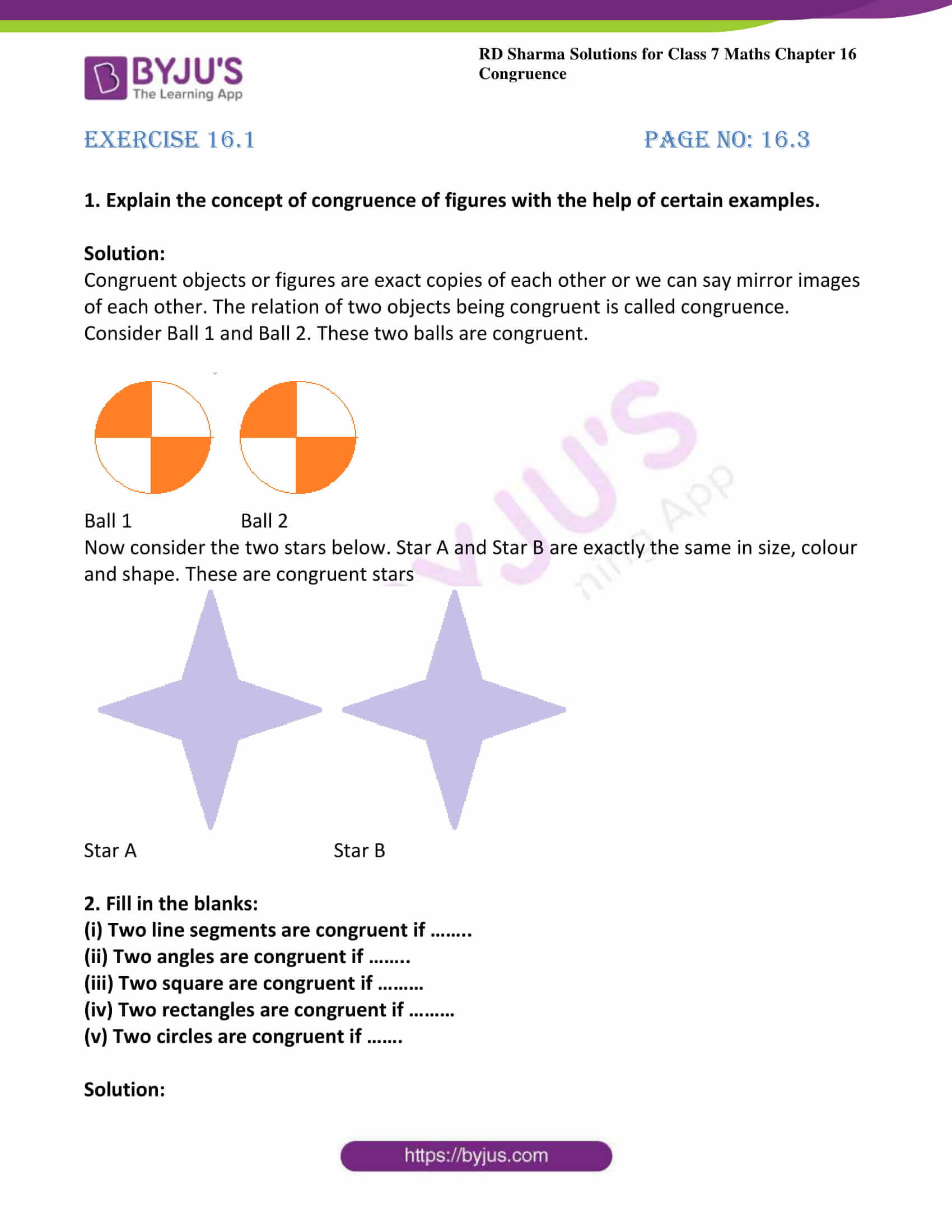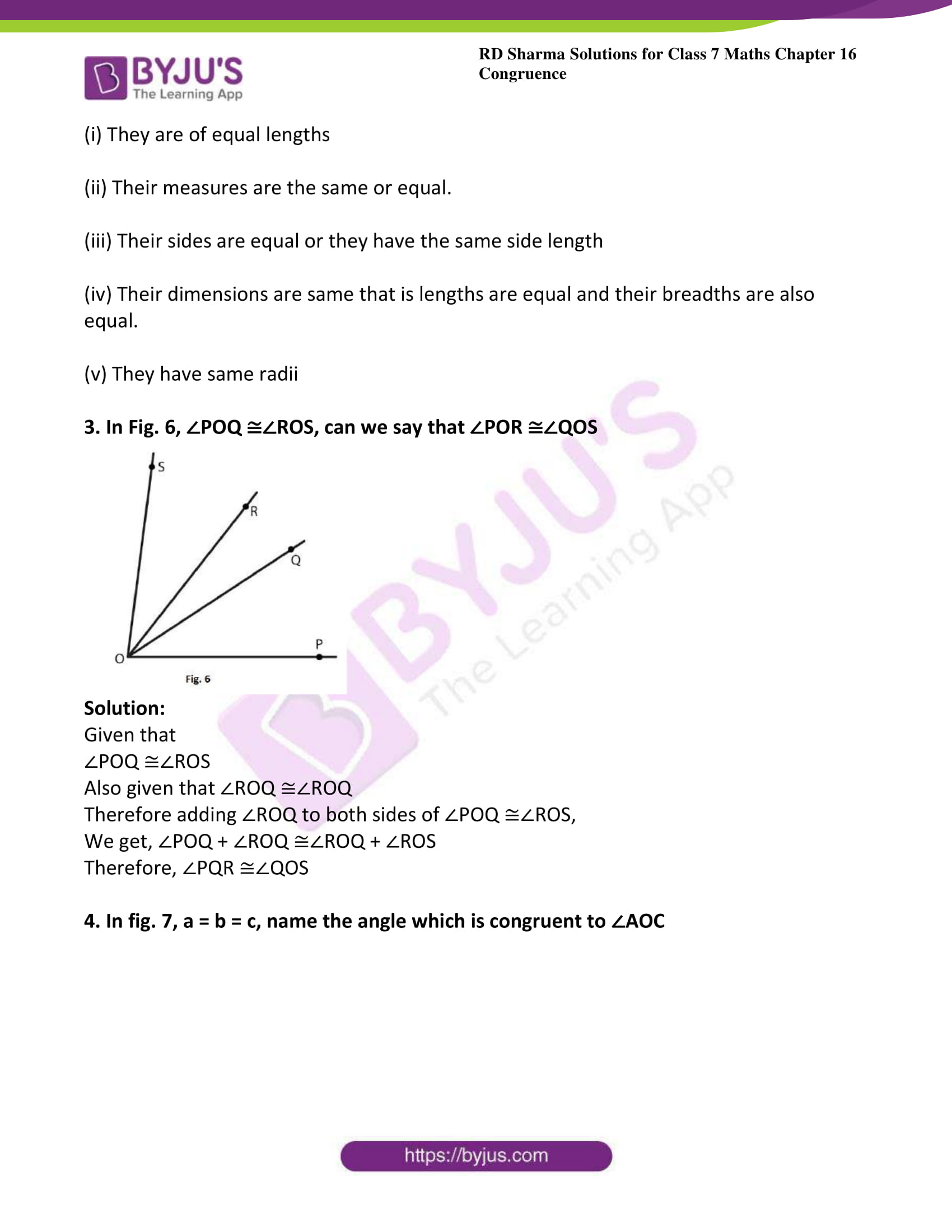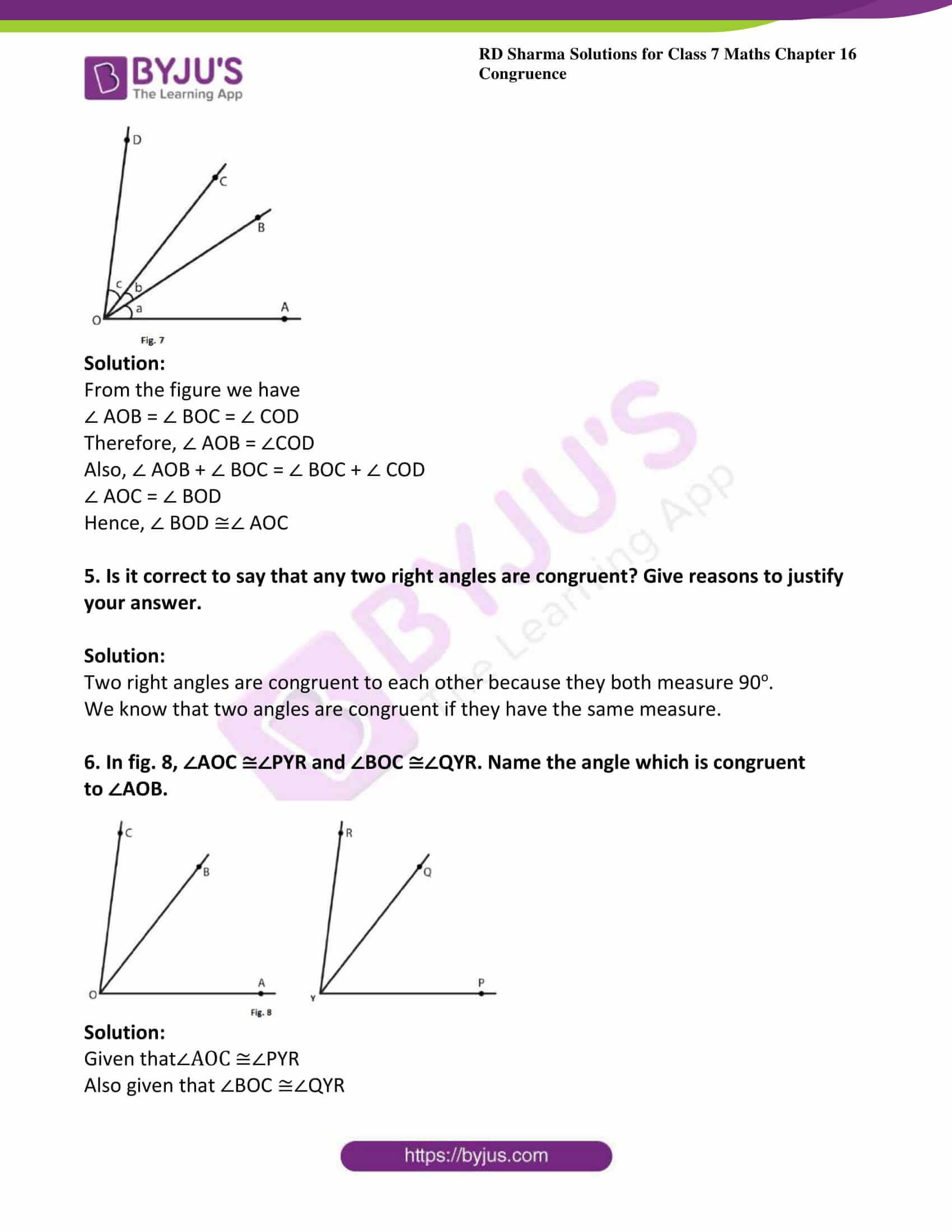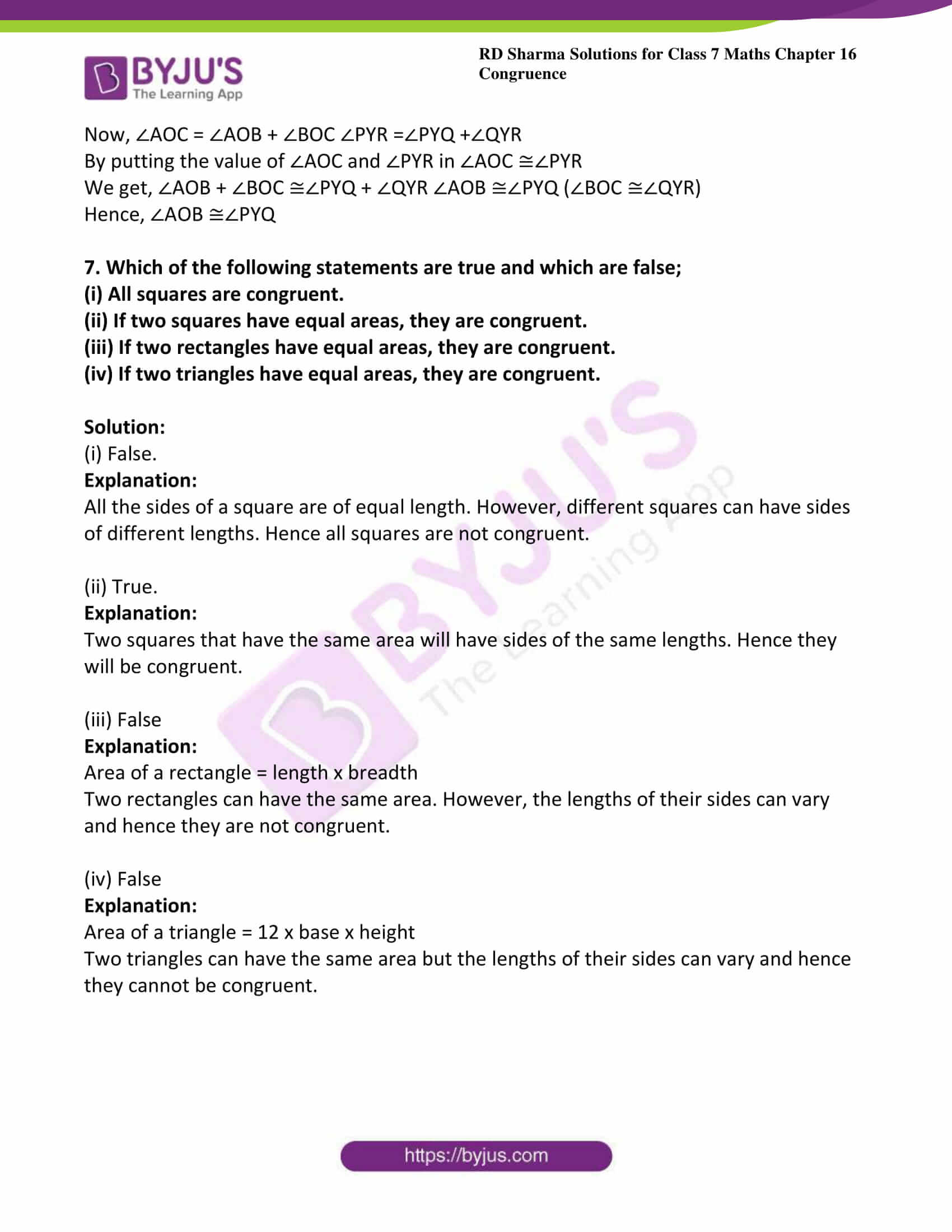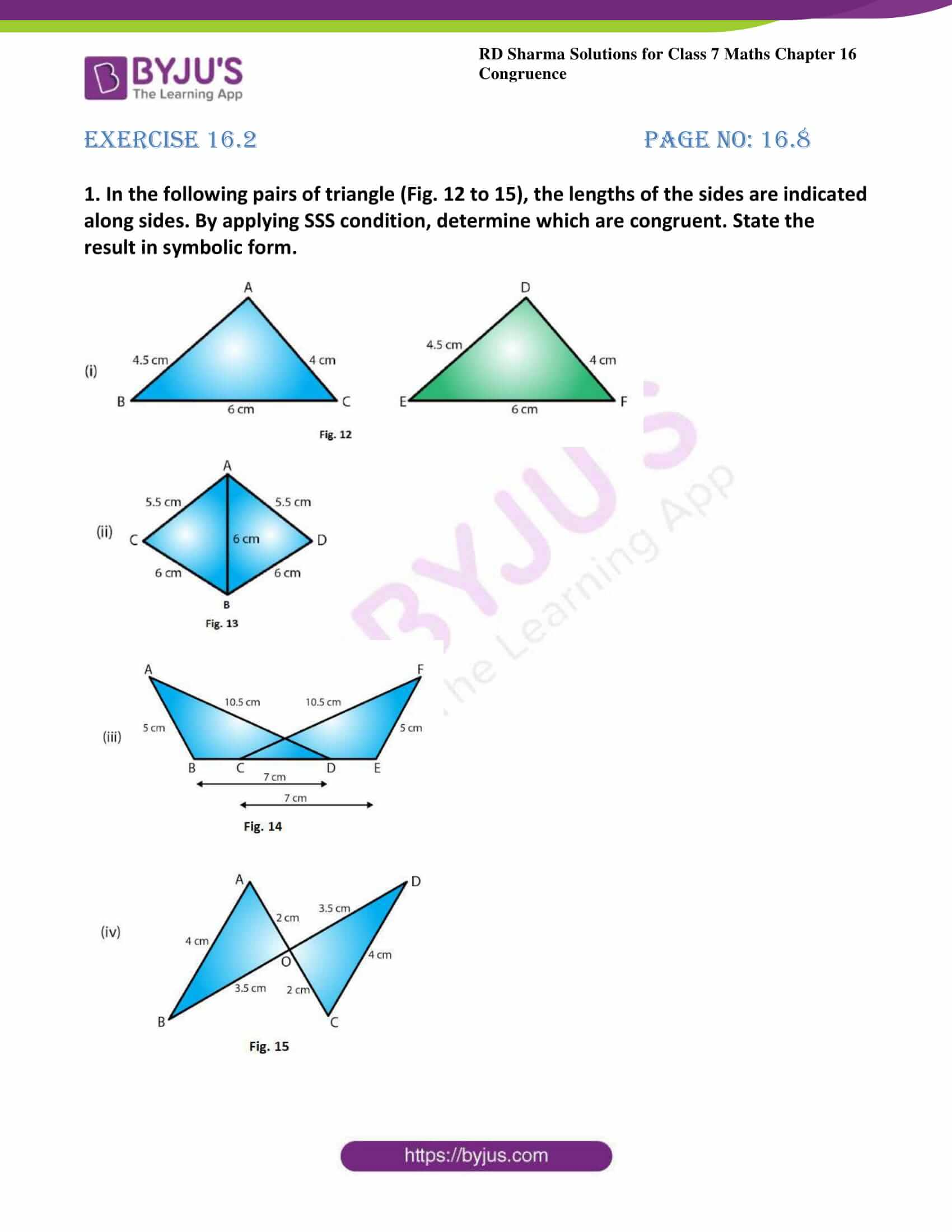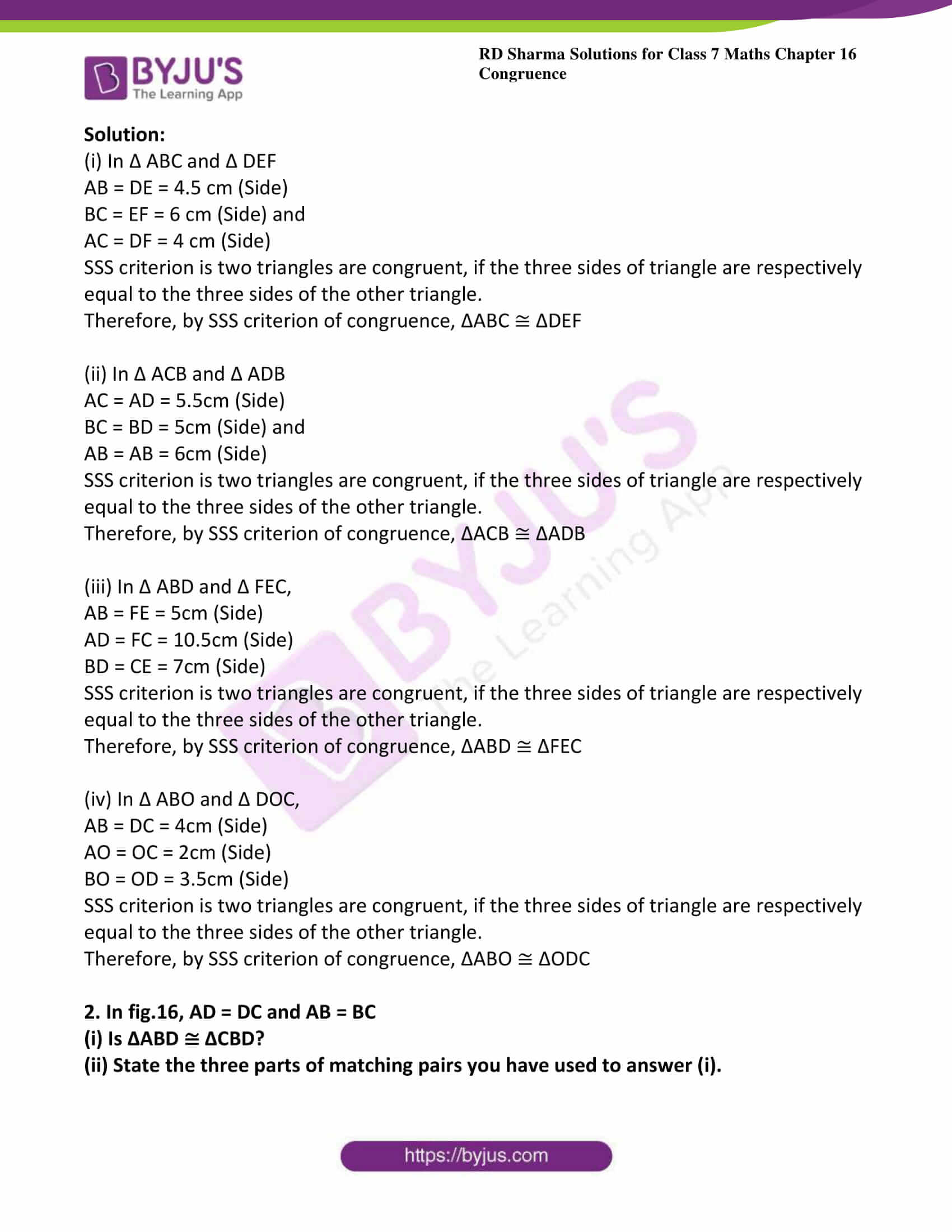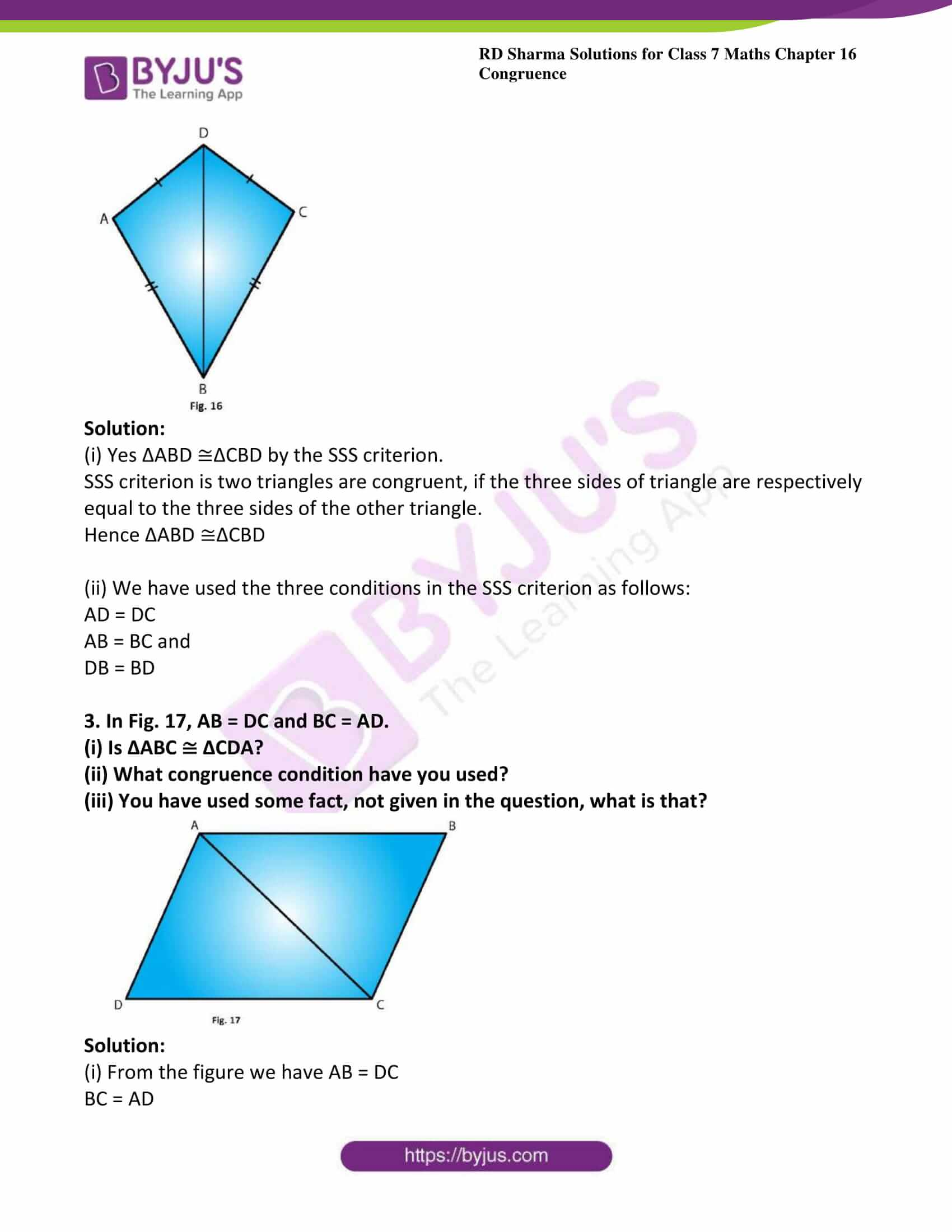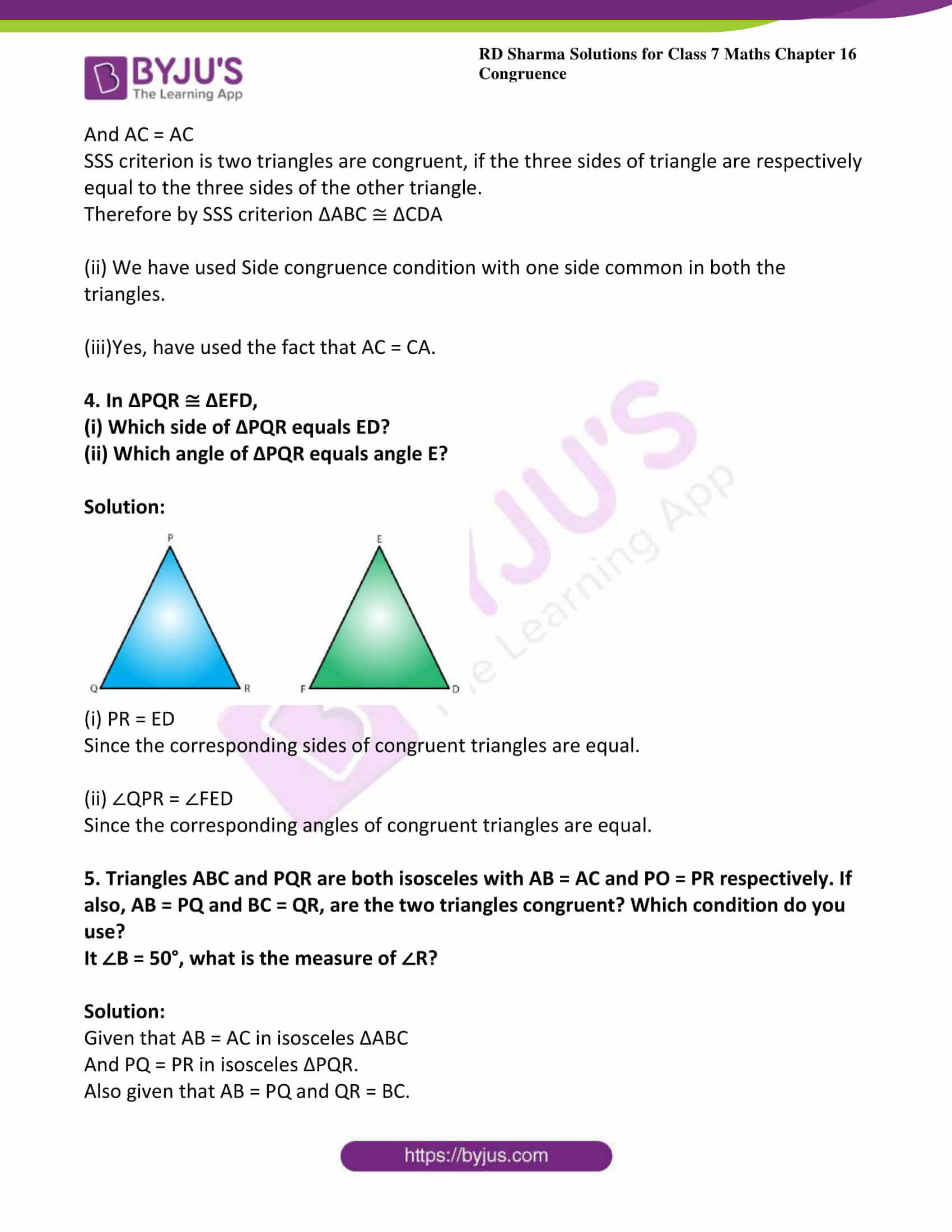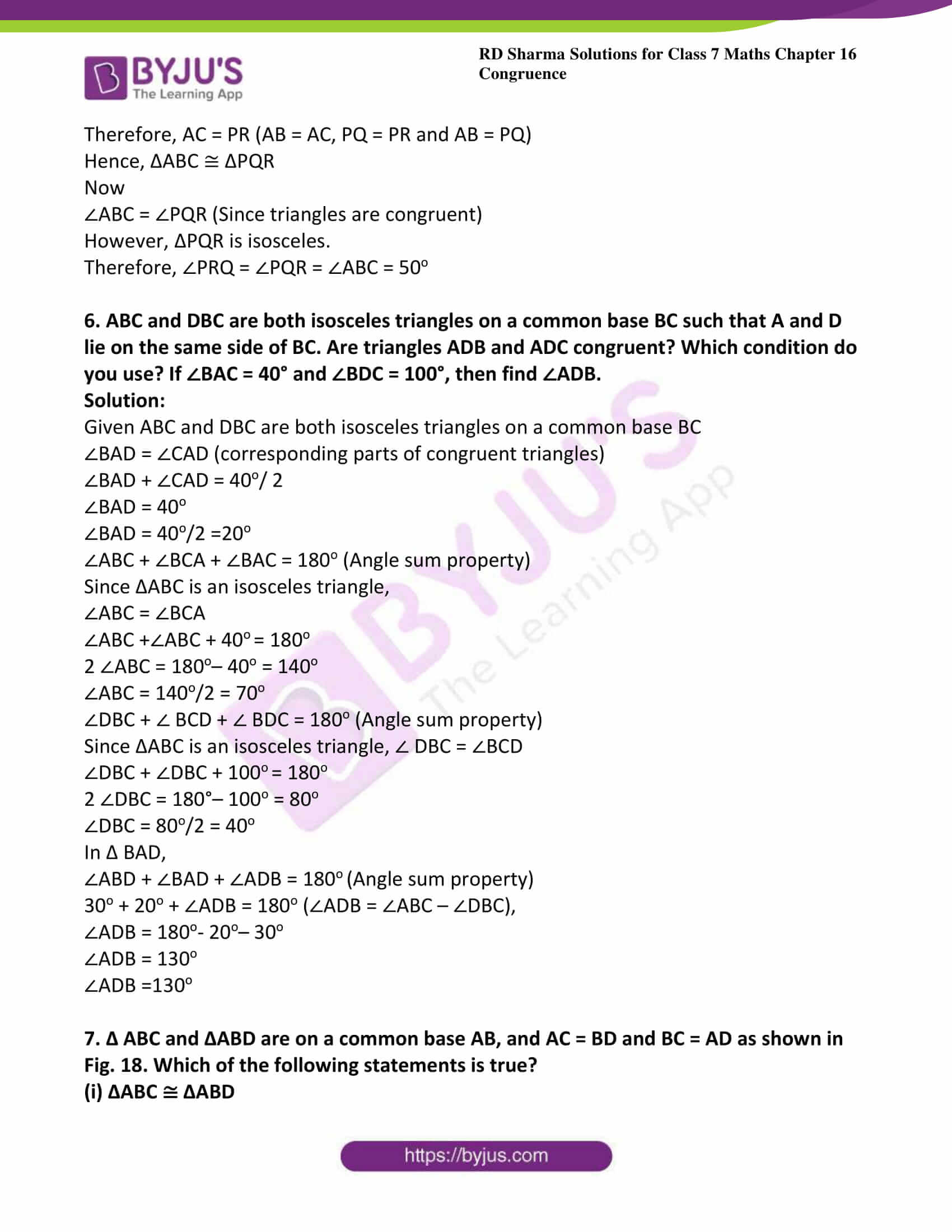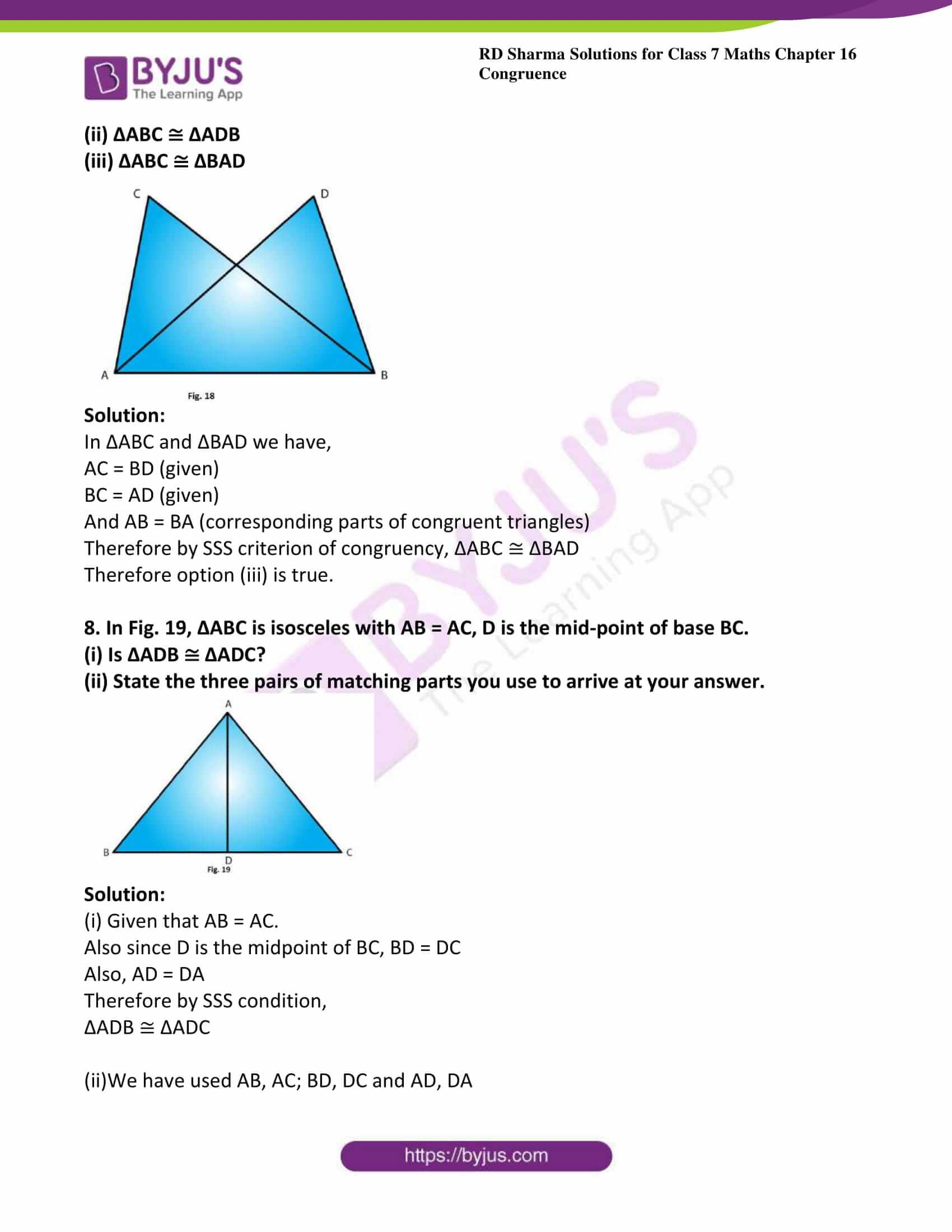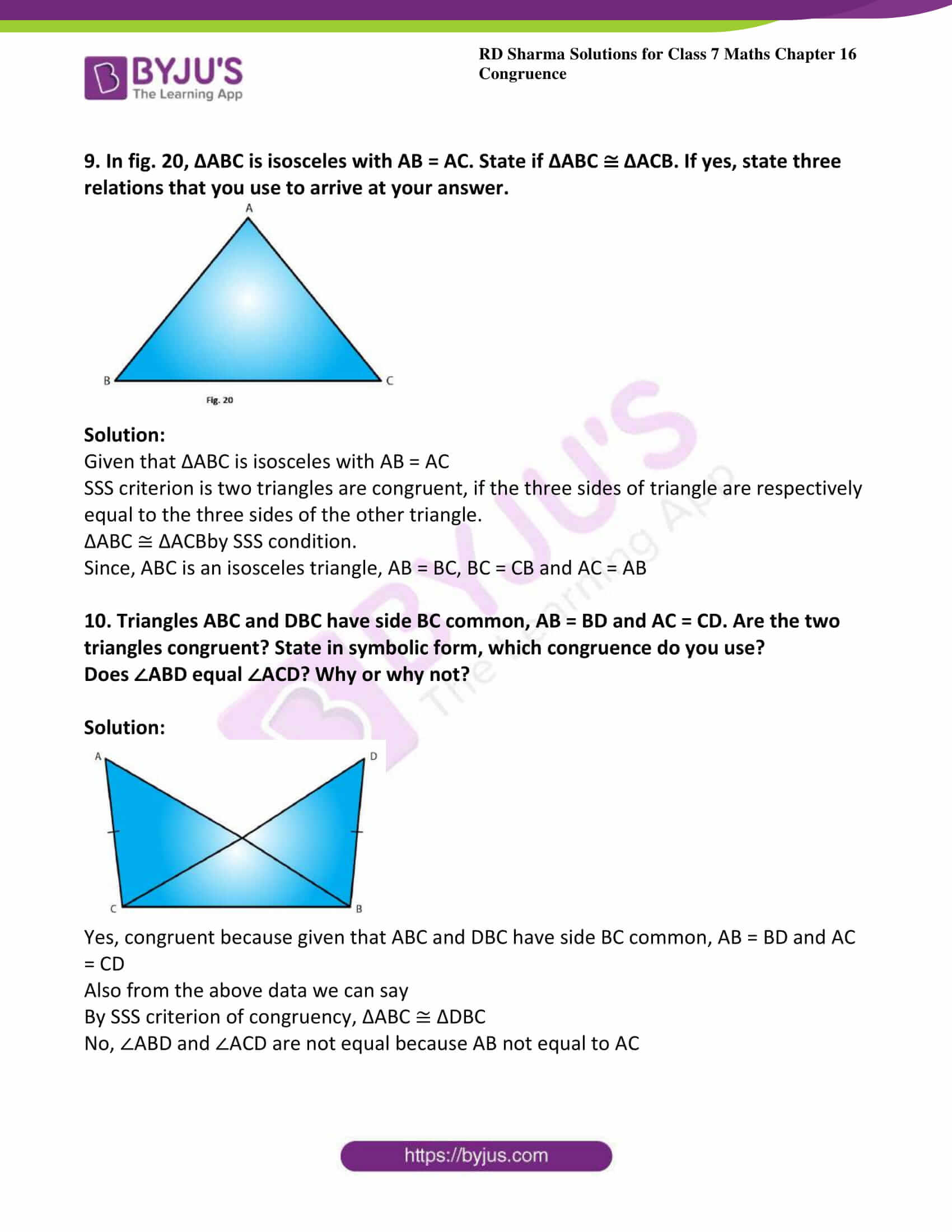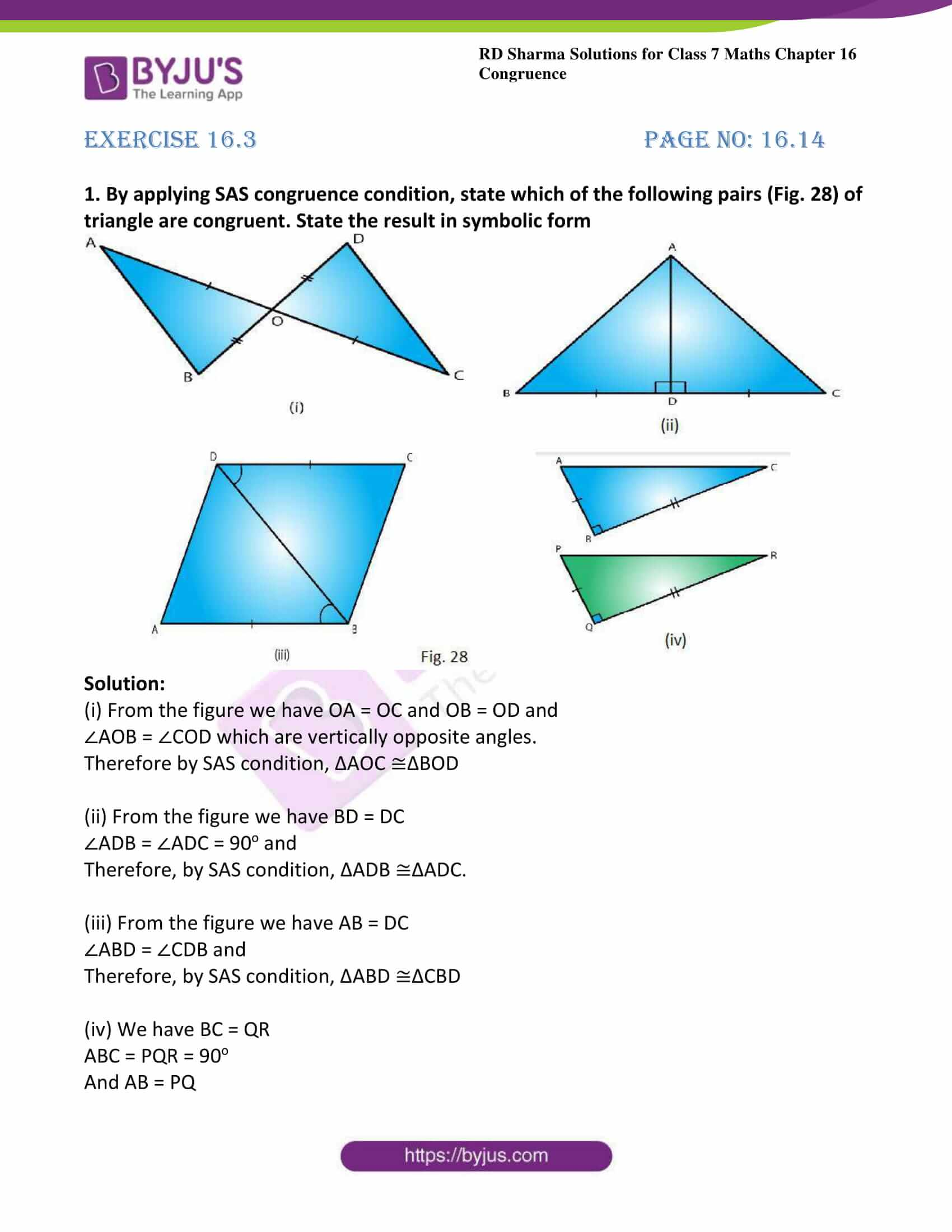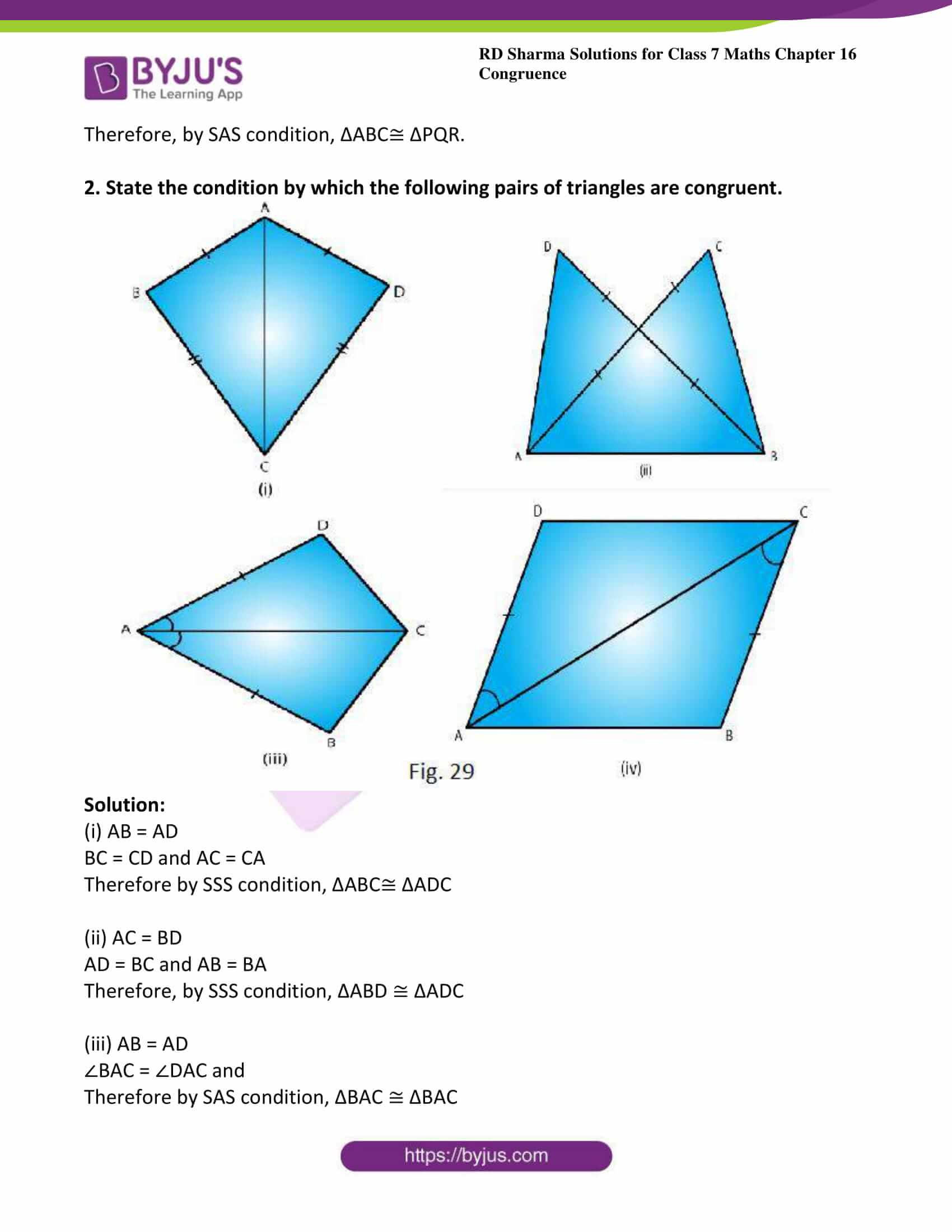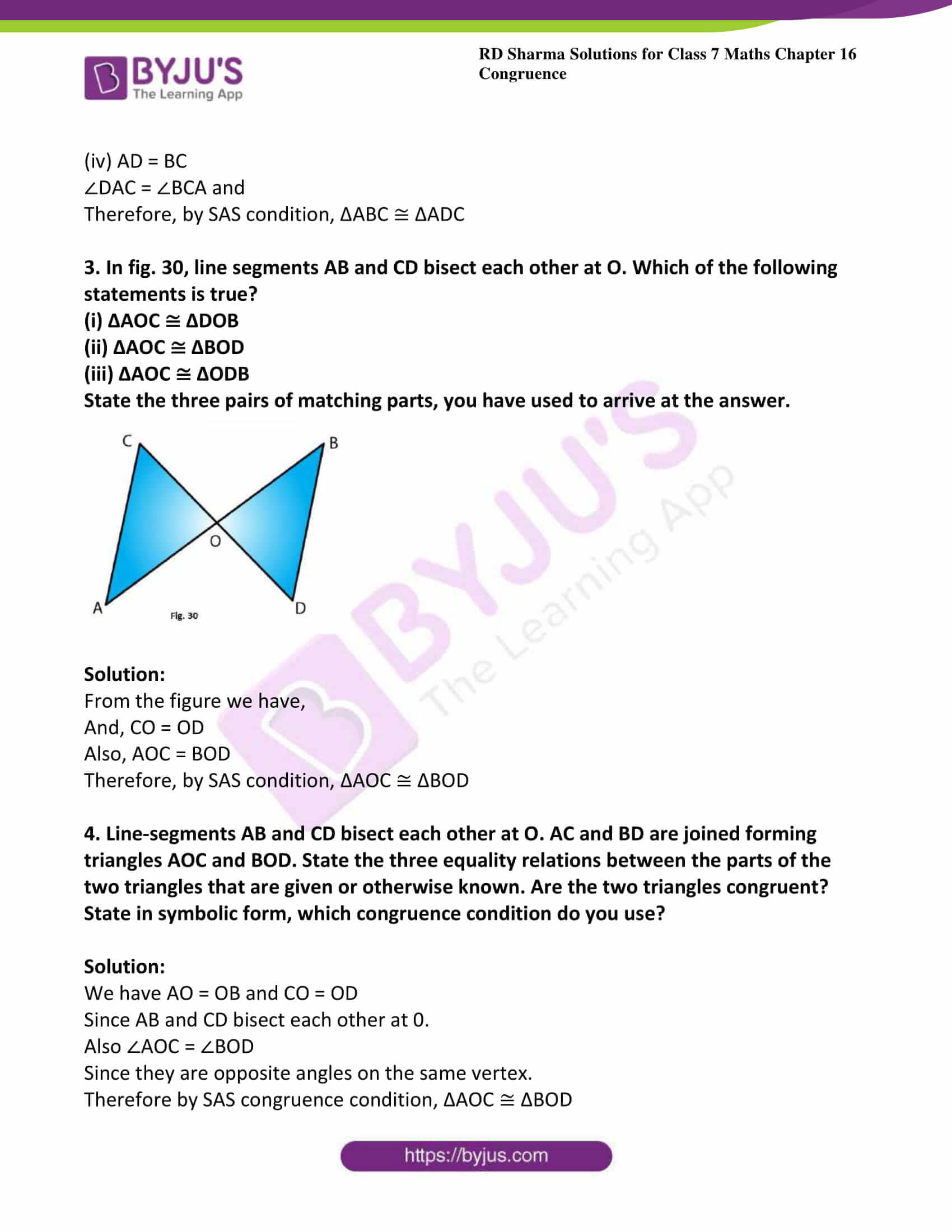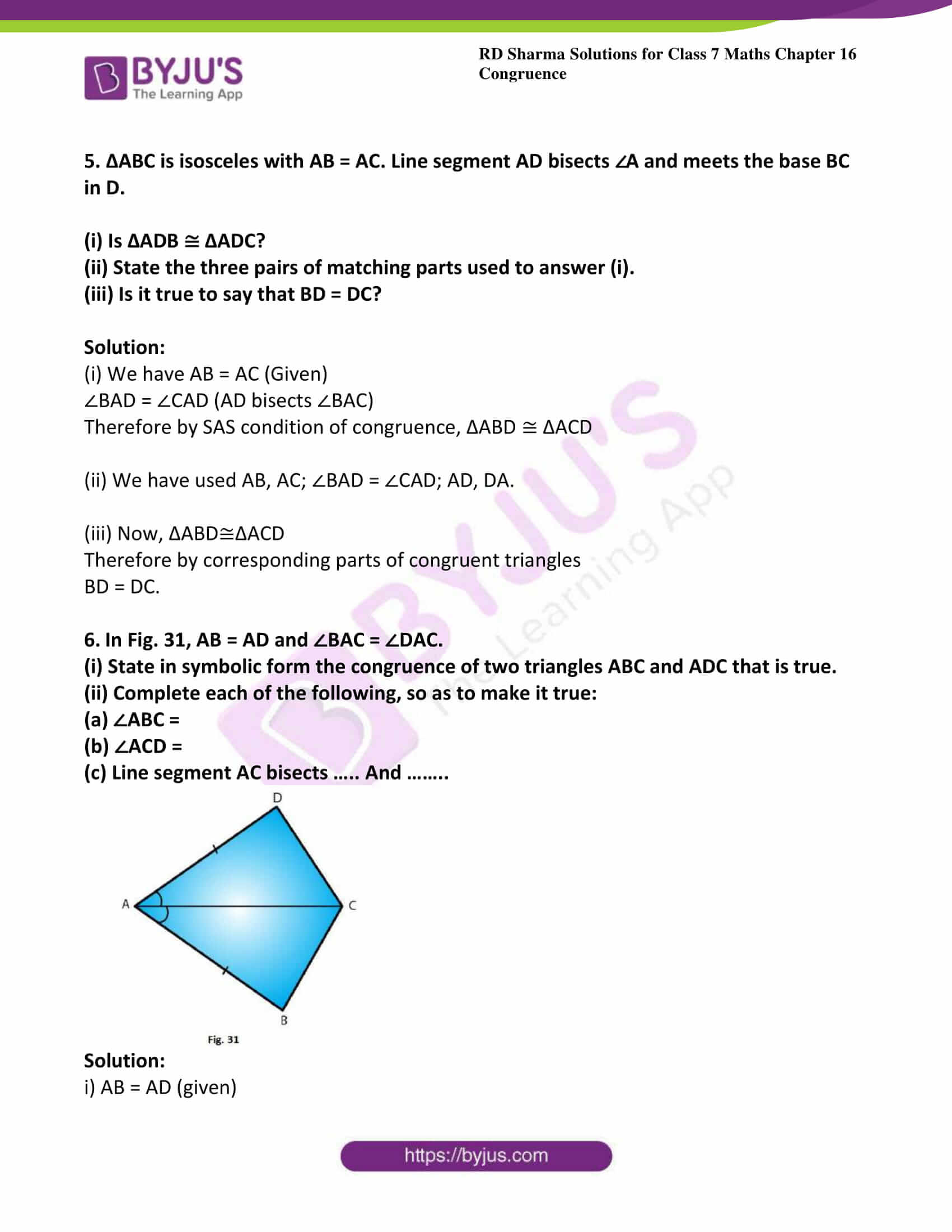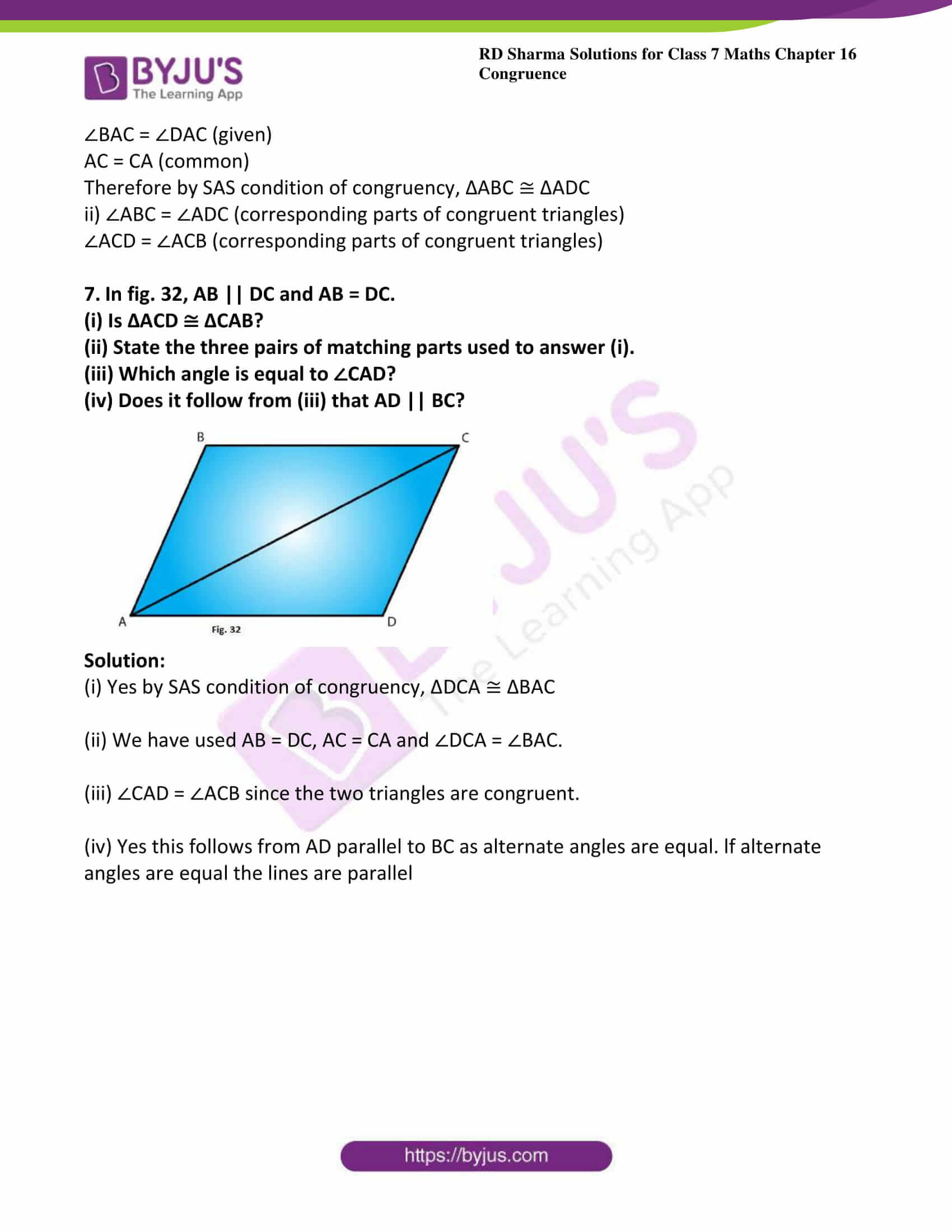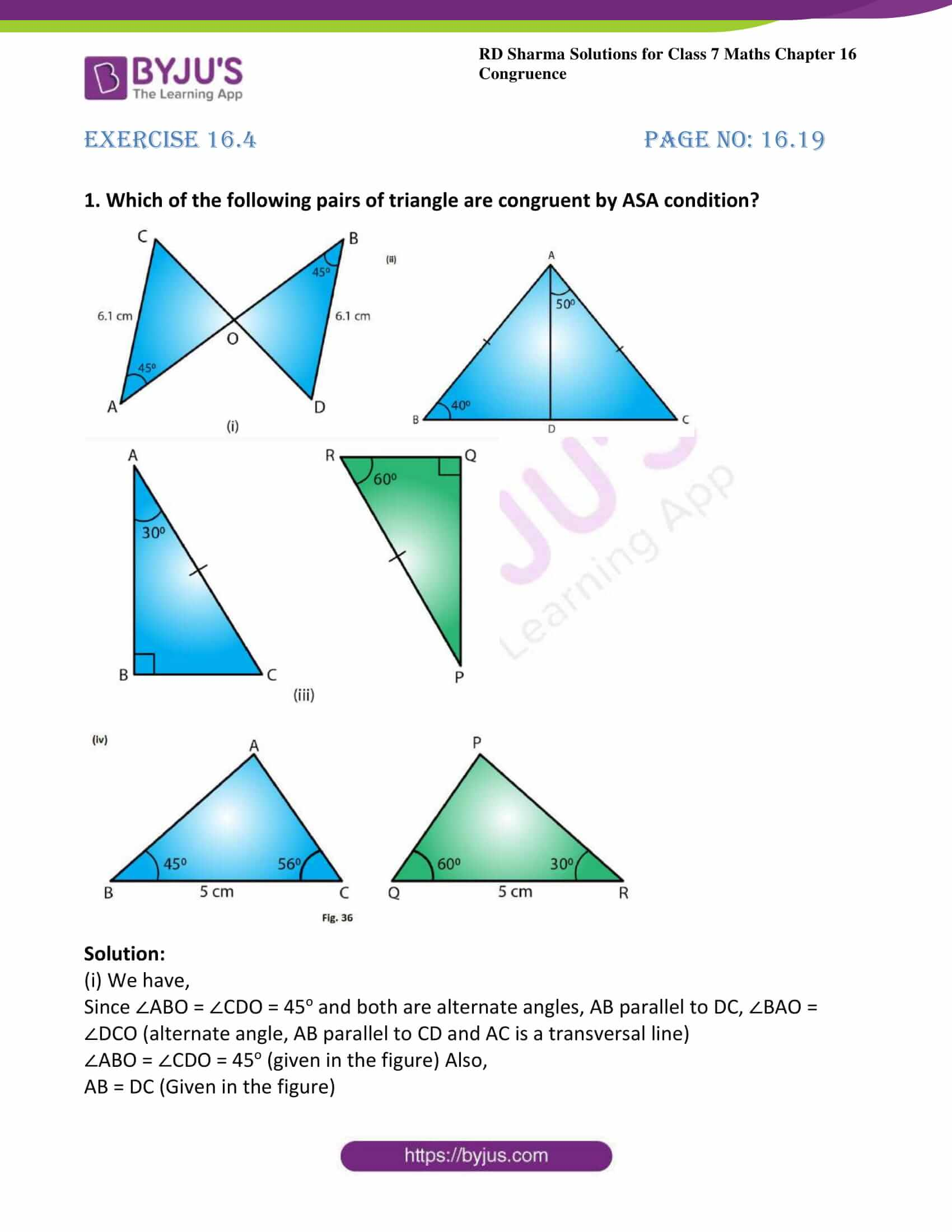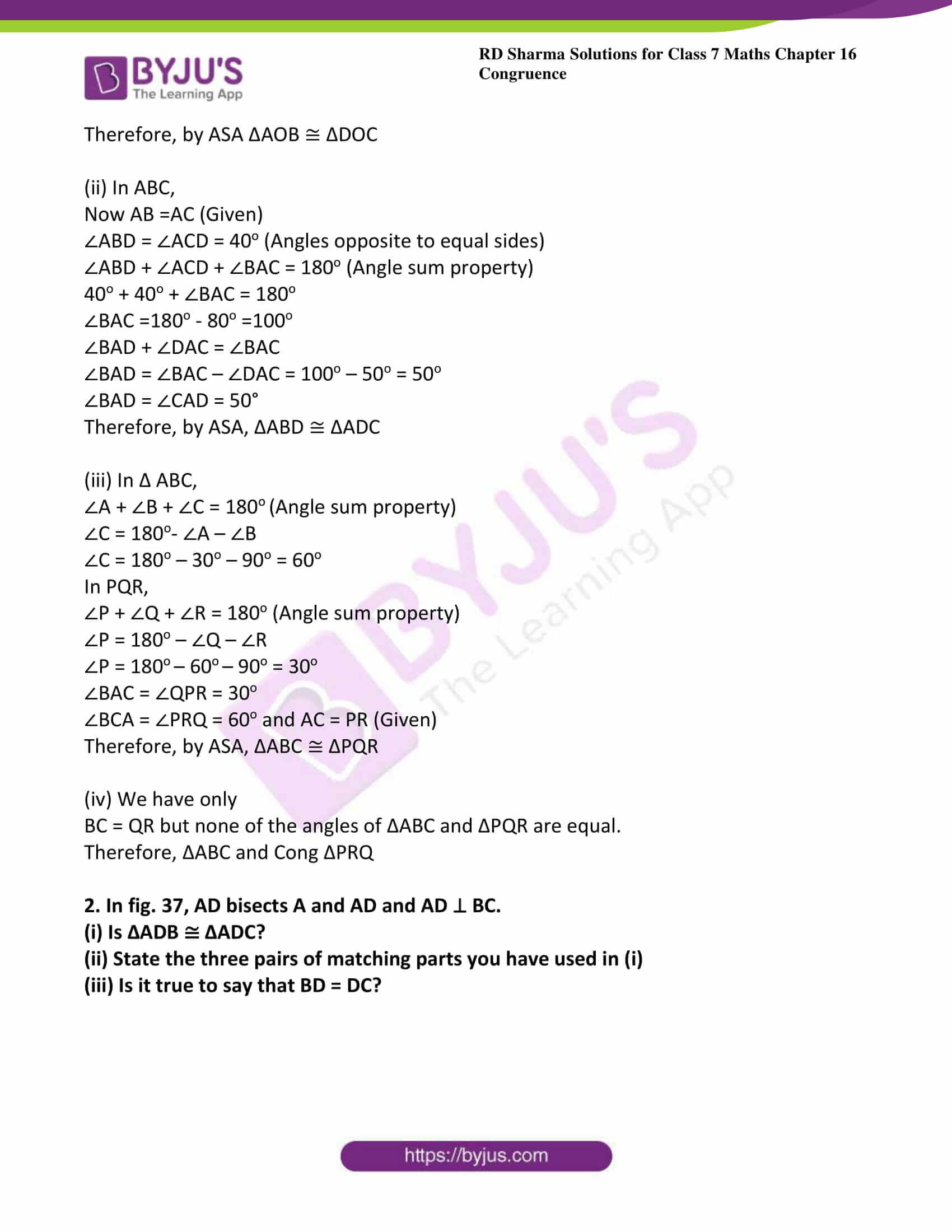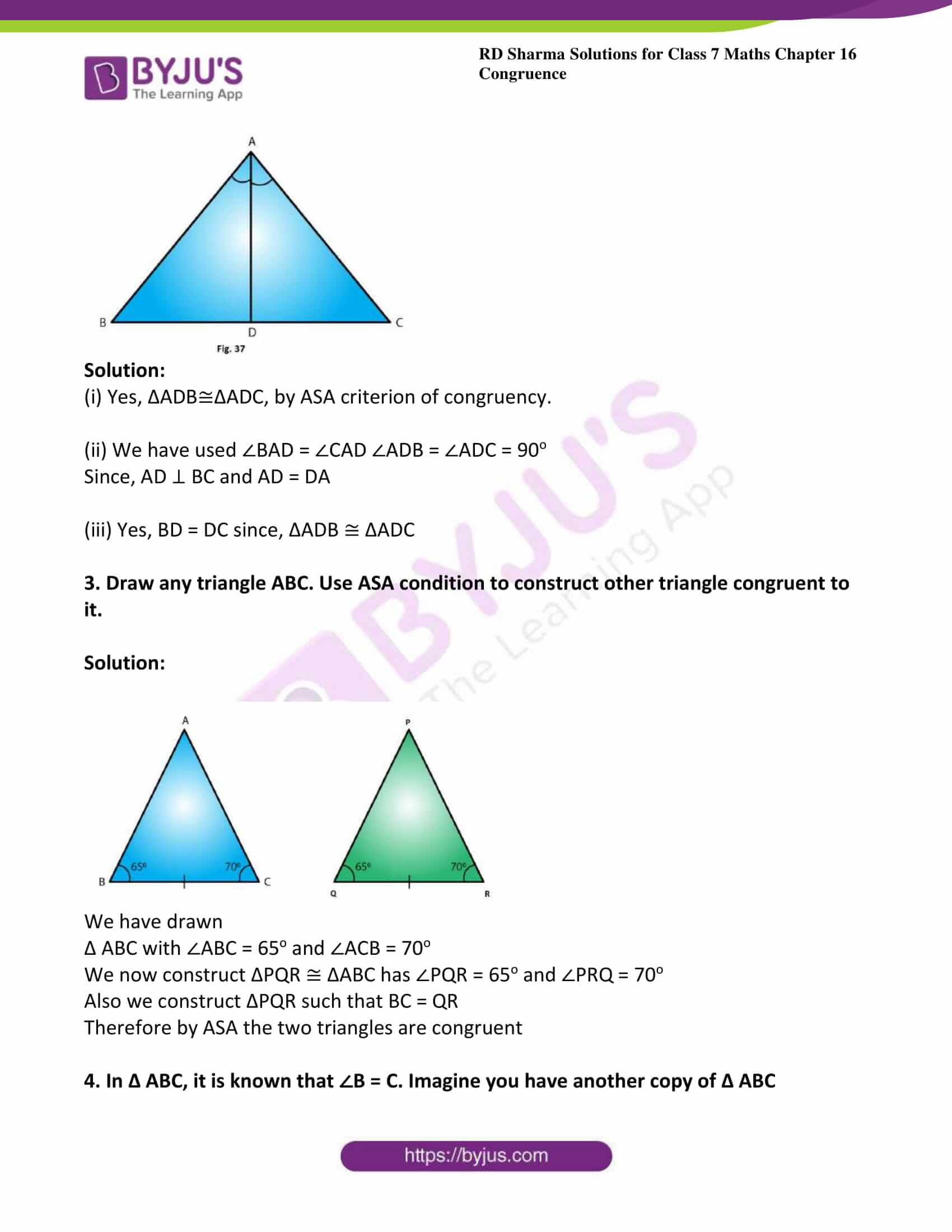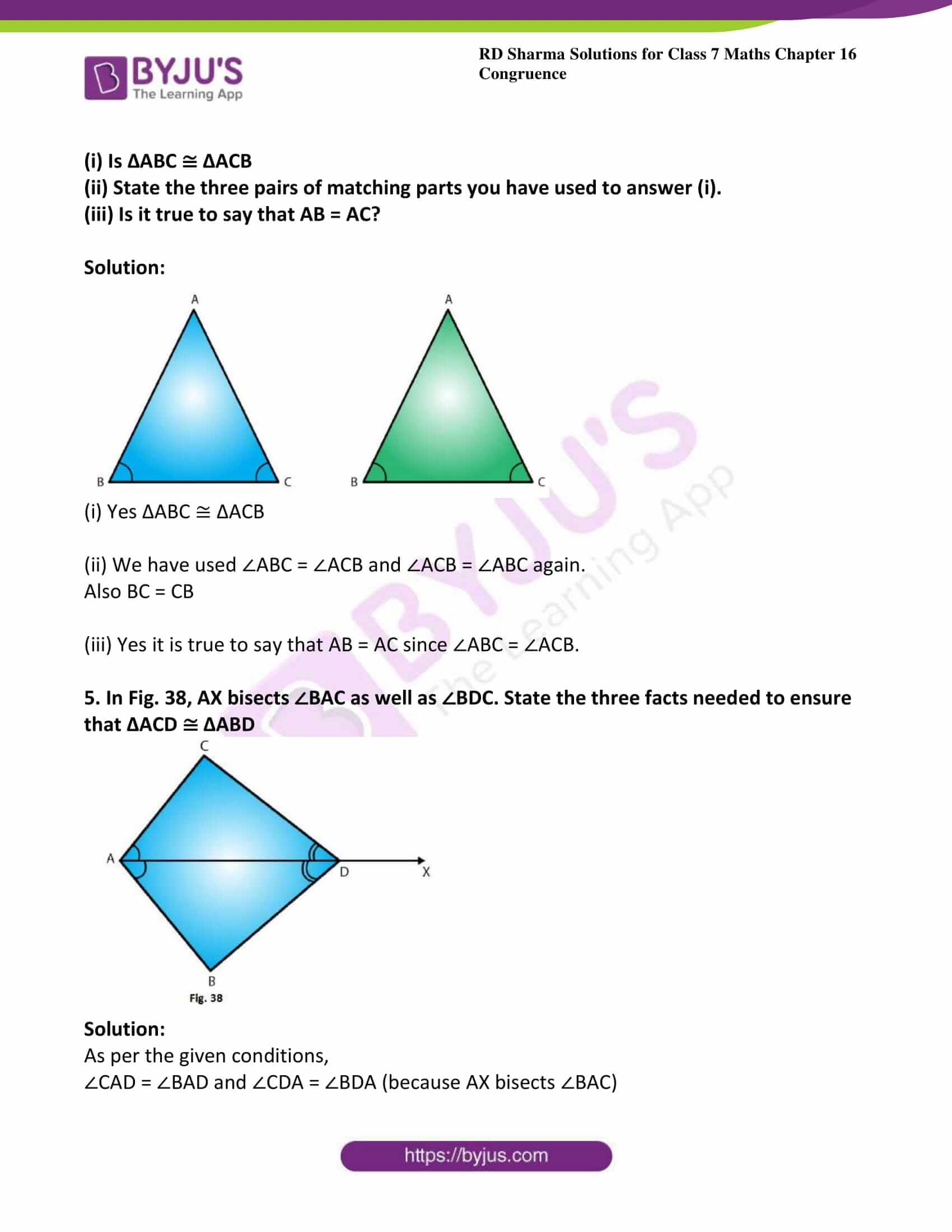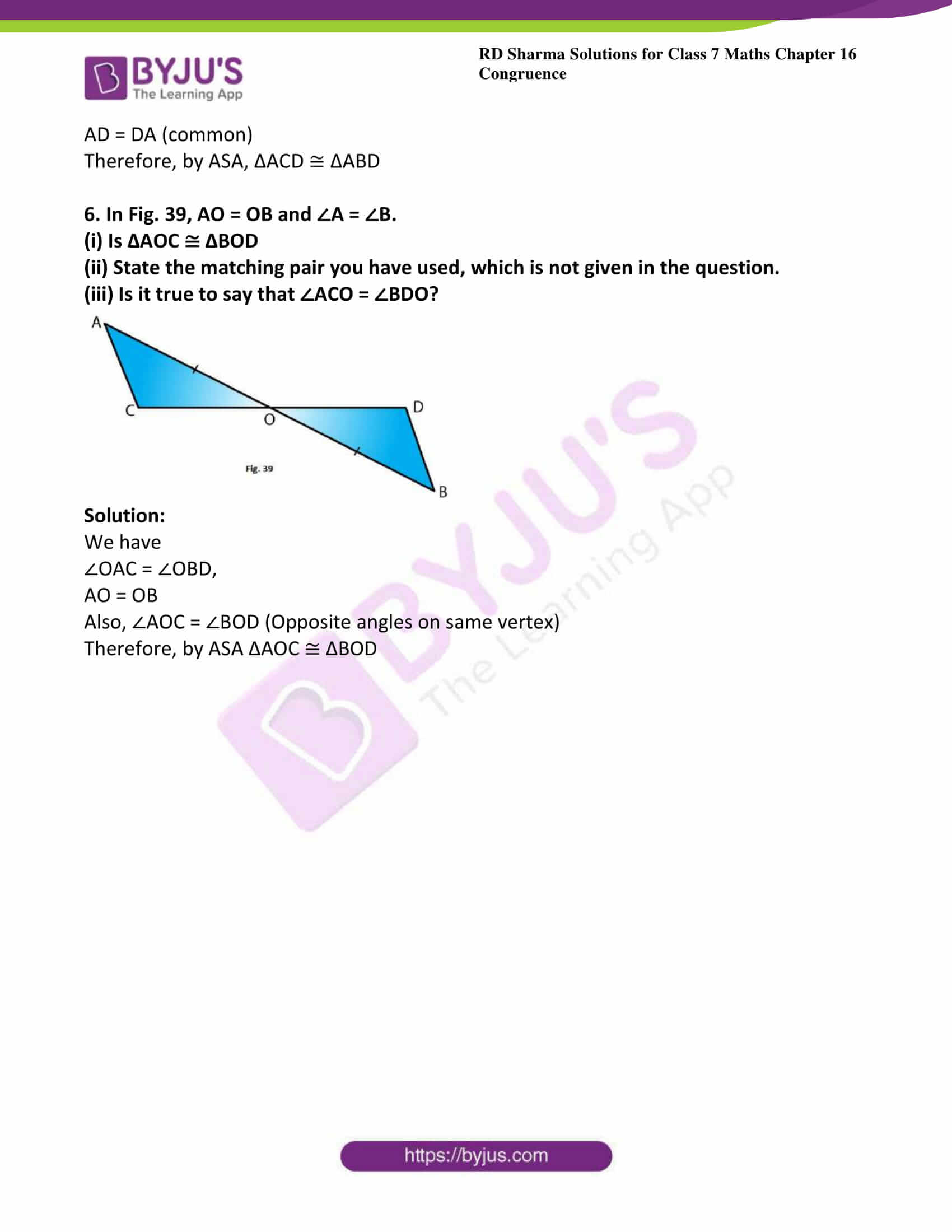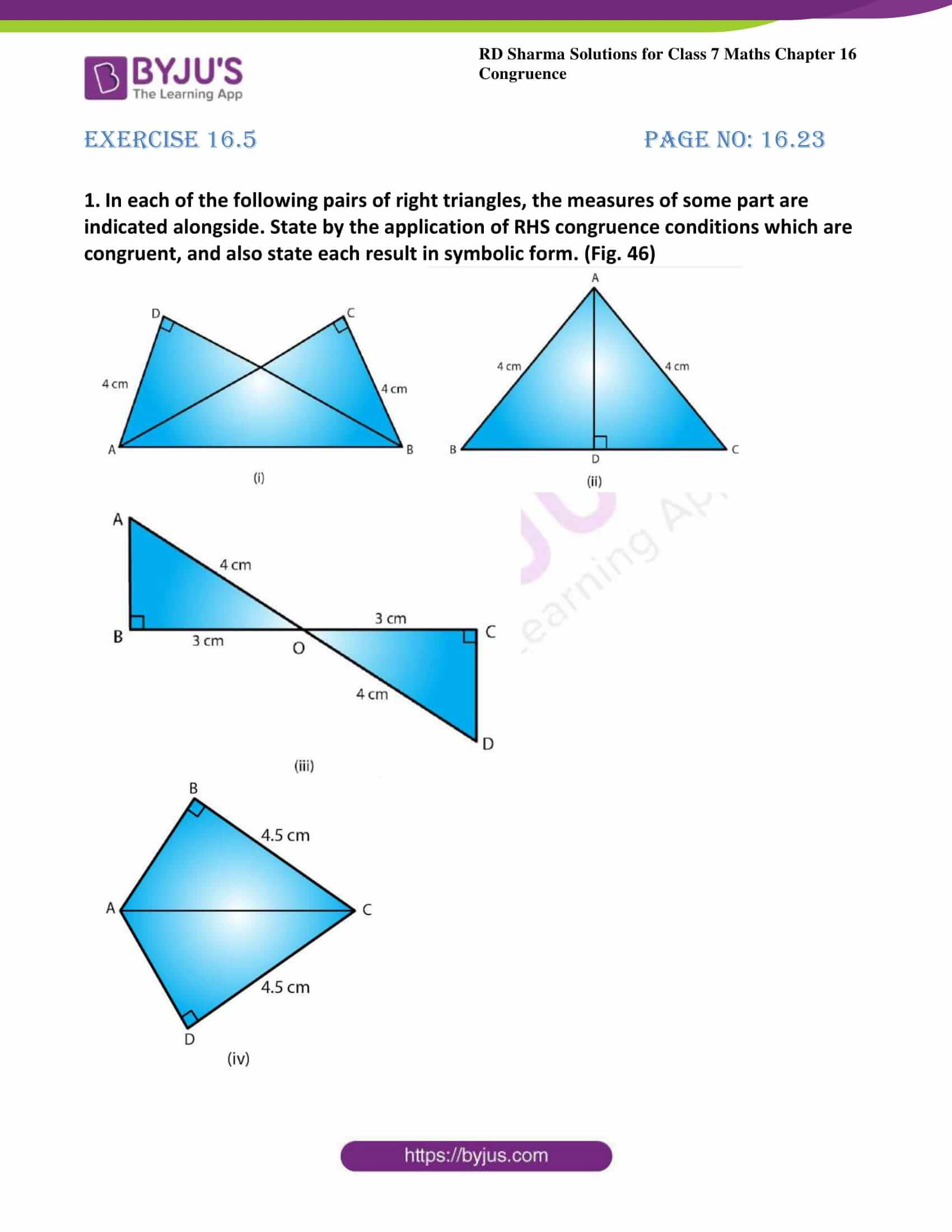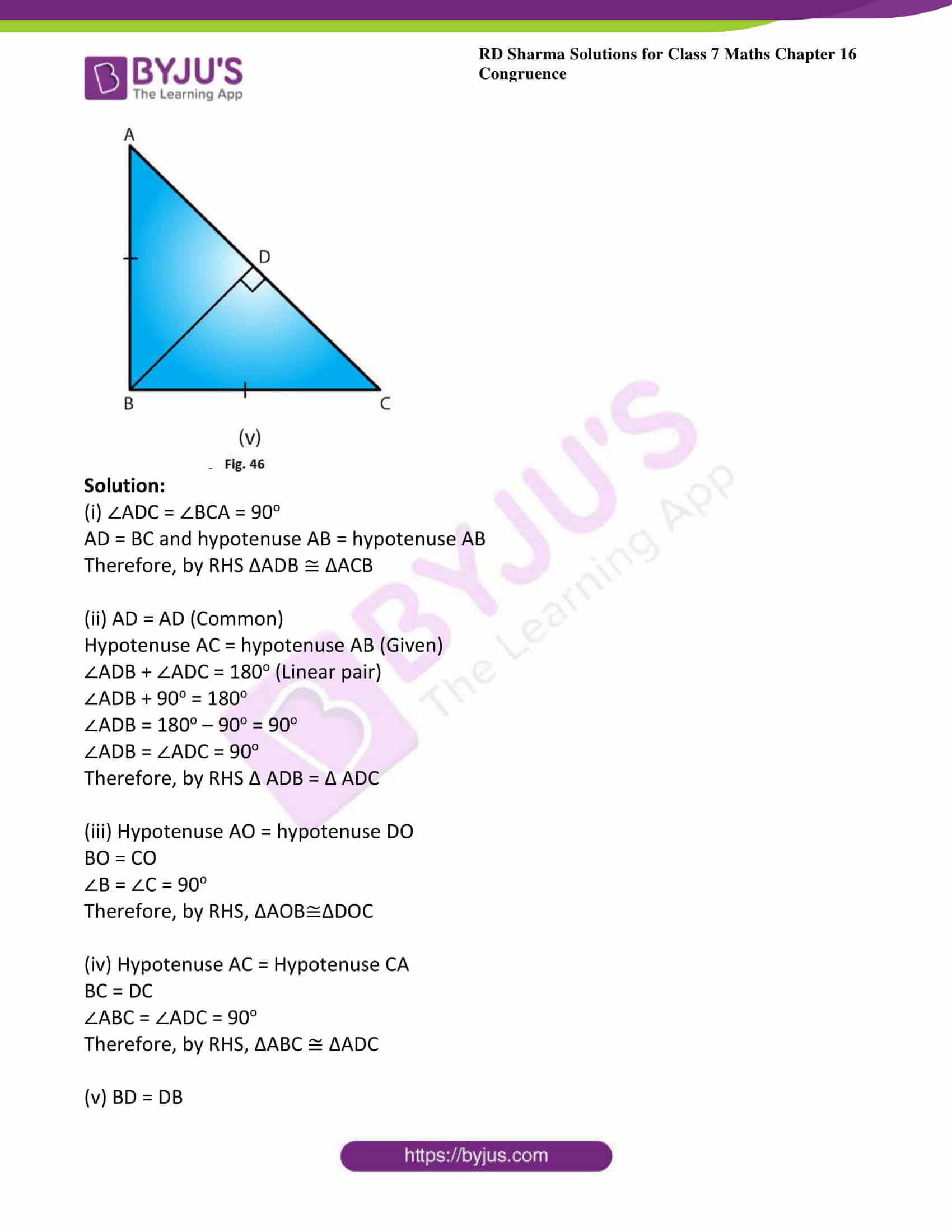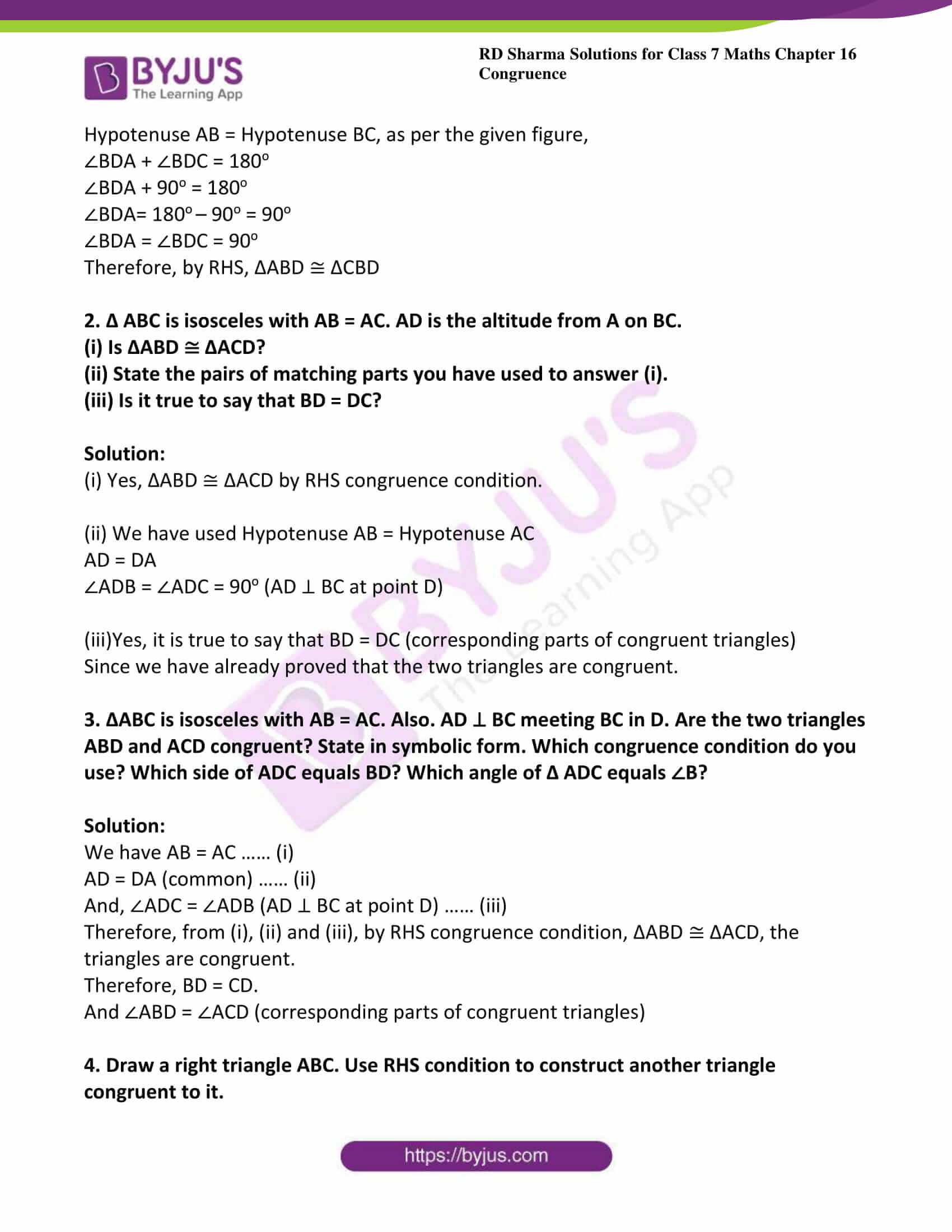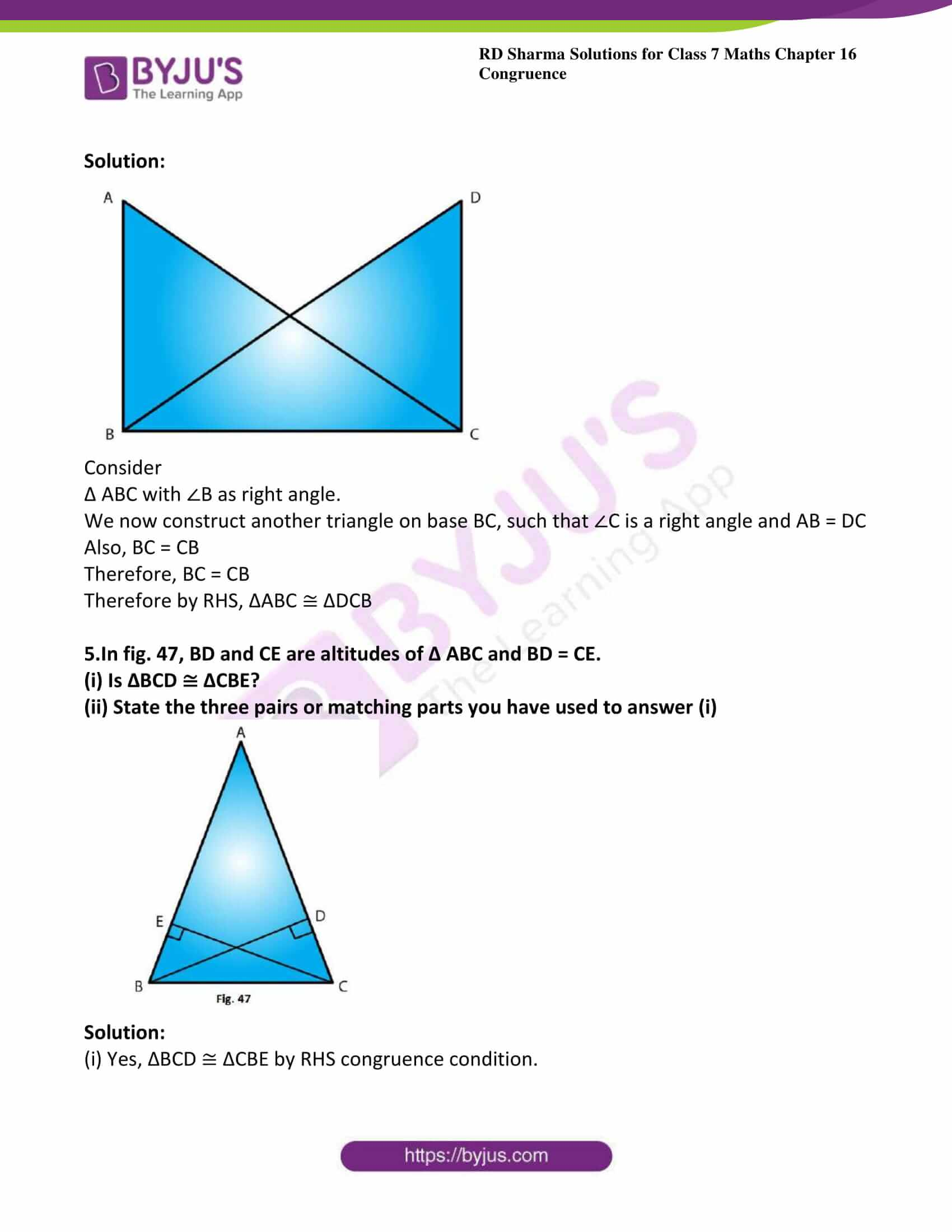### Access answers to Maths RD Sharma Solutions For Class 7 Chapter 16 – Congruence

Exercise 16.1 Page No: 16.3

1. Explain the concept of congruence of figures with the help of certain examples.

Solution:

Congruent objects or figures are exact copies of each other or we can say mirror images of each other. The relation of two objects being congruent is called congruence.

Consider Ball 1 and Ball 2. These two balls are congruent.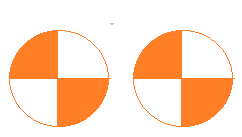Ball 1 Ball 2

Now consider the two stars below. Star A and Star B are exactly the same in size, colour and shape. These are congruent starsStar A Star B

2. Fill in the blanks:

(i) Two line segments are congruent if ……..

(ii) Two angles are congruent if ……..

(iii) Two square are congruent if ………

(iv) Two rectangles are congruent if ………

(v) Two circles are congruent if …….

Solution:

(i) They are of equal lengths

(ii) Their measures are the same or equal.

(iii) Their sides are equal or they have the same side length

(iv) Their dimensions are same that is lengths are equal and their breadths are also equal.

3. In Fig. 6, ∠POQ ≅∠ROS, can we say that ∠POR ≅∠QOS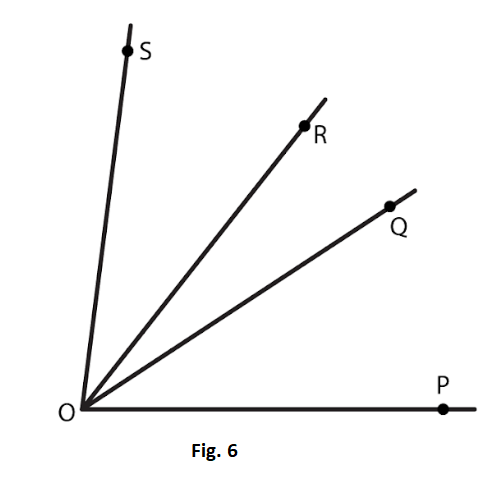Solution:

Given that

∠POQ ≅∠ROS

Also given that ∠ROQ ≅∠ROQ

Therefore adding ∠ROQ to both sides of ∠POQ ≅∠ROS,

We get, ∠POQ + ∠ROQ ≅∠ROQ + ∠ROS

Therefore, ∠PQR ≅∠QOS

4. In fig. 7, a = b = c, name the angle which is congruent to ∠AOC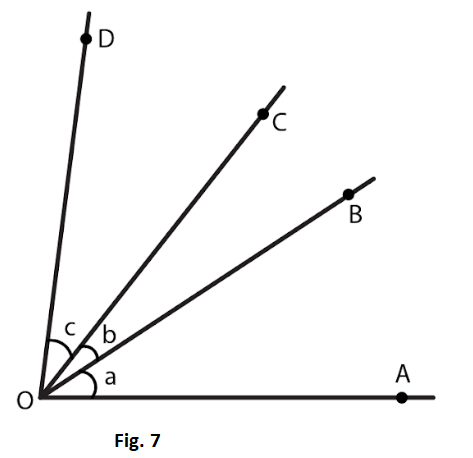Solution:

From the figure we have

∠ AOB = ∠ BOC = ∠ COD

Therefore, ∠ AOB = ∠COD

Also, ∠ AOB + ∠ BOC = ∠ BOC + ∠ COD

∠ AOC = ∠ BOD

Hence, ∠ BOD ≅∠ AOC

5. Is it correct to say that any two right angles are congruent? Give reasons to justify your answer.

Solution:

Two right angles are congruent to each other because they both measure 90o.

We know that two angles are congruent if they have the same measure.

6. In fig. 8, ∠AOC ≅∠PYR and ∠BOC ≅∠QYR. Name the angle which is congruent to ∠AOB.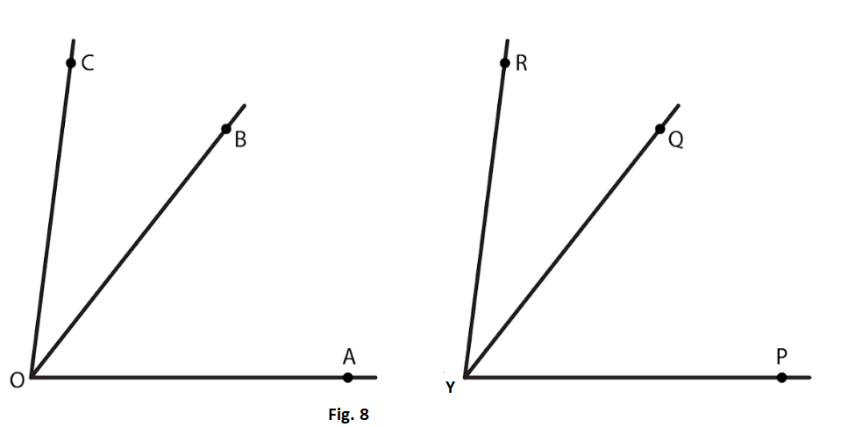Solution:

Given that∠AOC ≅∠PYR

Also given that ∠BOC ≅∠QYR

Now, ∠AOC = ∠AOB + ∠BOC ∠PYR =∠PYQ +∠QYR

By putting the value of ∠AOC and ∠PYR in ∠AOC ≅∠PYR

We get, ∠AOB + ∠BOC ≅∠PYQ + ∠QYR ∠AOB ≅∠PYQ (∠BOC ≅∠QYR)

Hence, ∠AOB ≅∠PYQ

7. Which of the following statements are true and which are false;

(i) All squares are congruent.

(ii) If two squares have equal areas, they are congruent.

(iii) If two rectangles have equal areas, they are congruent.

(iv) If two triangles have equal areas, they are congruent.

Solution:

(i) False.

Explanation:

All the sides of a square are of equal length. However, different squares can have sides of different lengths. Hence all squares are not congruent.

(ii) True.

Explanation:

Two squares that have the same area will have sides of the same lengths. Hence they will be congruent.

(iii) False

Explanation:

Area of a rectangle = length x breadth

Two rectangles can have the same area. However, the lengths of their sides can vary and hence they are not congruent.

(iv) False

Explanation:

Area of a triangle = 12 x base x height

Two triangles can have the same area but the lengths of their sides can vary and hence they cannot be congruent.

Exercise 16.2 Page No: 16.8

1. In the following pairs of triangle (Fig. 12 to 15), the lengths of the sides are indicated along sides. By applying SSS condition, determine which are congruent. State the result in symbolic form.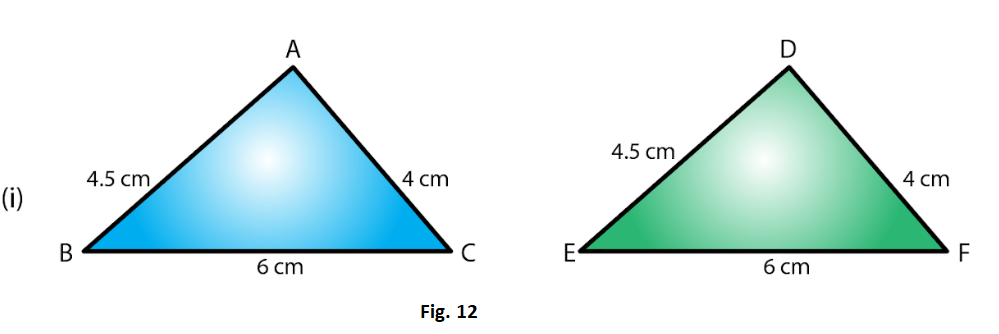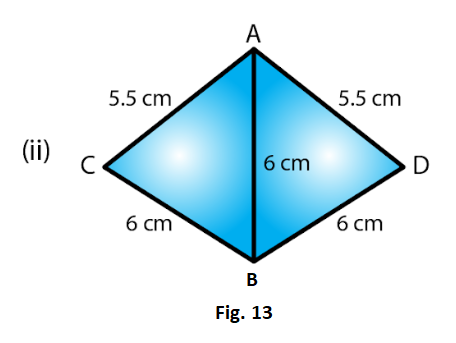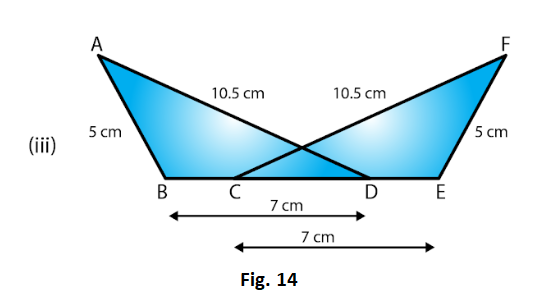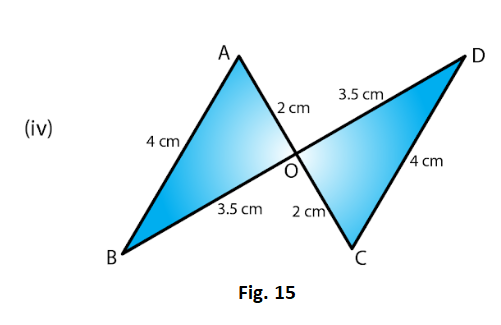Solution:

(i) In Δ ABC and Δ DEF

AB = DE = 4.5 cm (Side)

BC = EF = 6 cm (Side) and

AC = DF = 4 cm (Side)

SSS criterion is two triangles are congruent, if the three sides of triangle are respectively equal to the three sides of the other triangle.

Therefore, by SSS criterion of congruence, ΔABC ≅ ΔDEF

(ii) In Δ ACB and Δ ADB

AC = AD = 5.5cm (Side)

BC = BD = 5cm (Side) and

AB = AB = 6cm (Side)

SSS criterion is two triangles are congruent, if the three sides of triangle are respectively equal to the three sides of the other triangle.

Therefore, by SSS criterion of congruence, ΔACB ≅ ΔADB

(iii) In Δ ABD and Δ FEC,

AB = FE = 5cm (Side)

AD = FC = 10.5cm (Side)

BD = CE = 7cm (Side)

SSS criterion is two triangles are congruent, if the three sides of triangle are respectively equal to the three sides of the other triangle.

Therefore, by SSS criterion of congruence, ΔABD ≅ ΔFEC

(iv) In Δ ABO and Δ DOC,

AB = DC = 4cm (Side)

AO = OC = 2cm (Side)

BO = OD = 3.5cm (Side)

SSS criterion is two triangles are congruent, if the three sides of triangle are respectively equal to the three sides of the other triangle.

Therefore, by SSS criterion of congruence, ΔABO ≅ ΔODC

2. In fig.16, AD = DC and AB = BC

(i) Is ΔABD ≅ ΔCBD?

(ii) State the three parts of matching pairs you have used to answer (i).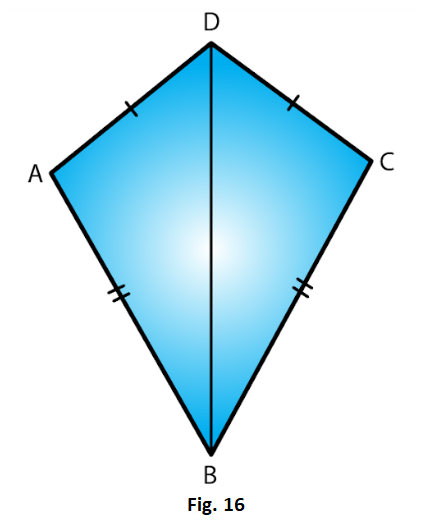Solution:

(i) Yes ΔABD ≅ΔCBD by the SSS criterion.

SSS criterion is two triangles are congruent, if the three sides of triangle are respectively equal to the three sides of the other triangle.

Hence ΔABD ≅ΔCBD

(ii) We have used the three conditions in the SSS criterion as follows:

AB = BC and

DB = BD

3. In Fig. 17, AB = DC and BC = AD.

(i) Is ΔABC ≅ ΔCDA?

(ii) What congruence condition have you used?

(iii) You have used some fact, not given in the question, what is that?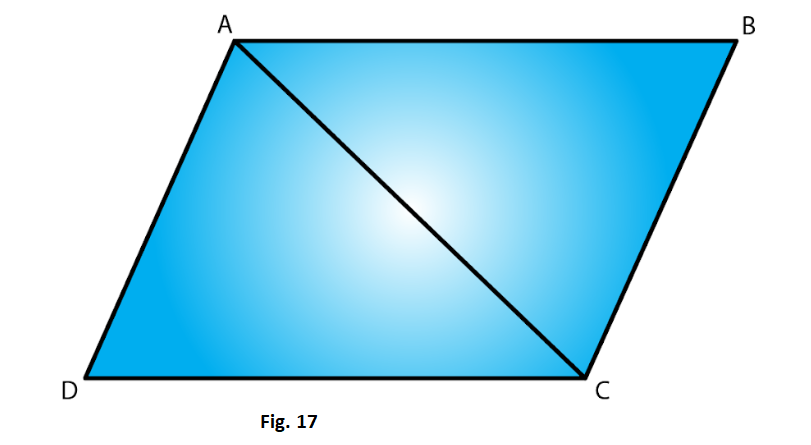Solution:

(i) From the figure we have AB = DC

And AC = AC

SSS criterion is two triangles are congruent, if the three sides of triangle are respectively equal to the three sides of the other triangle.

Therefore by SSS criterion ΔABC ≅ ΔCDA

(ii) We have used Side congruence condition with one side common in both the triangles.

(iii)Yes, have used the fact that AC = CA.

4. In ΔPQR ≅ ΔEFD,

(i) Which side of ΔPQR equals ED?

(ii) Which angle of ΔPQR equals angle E?

Solution: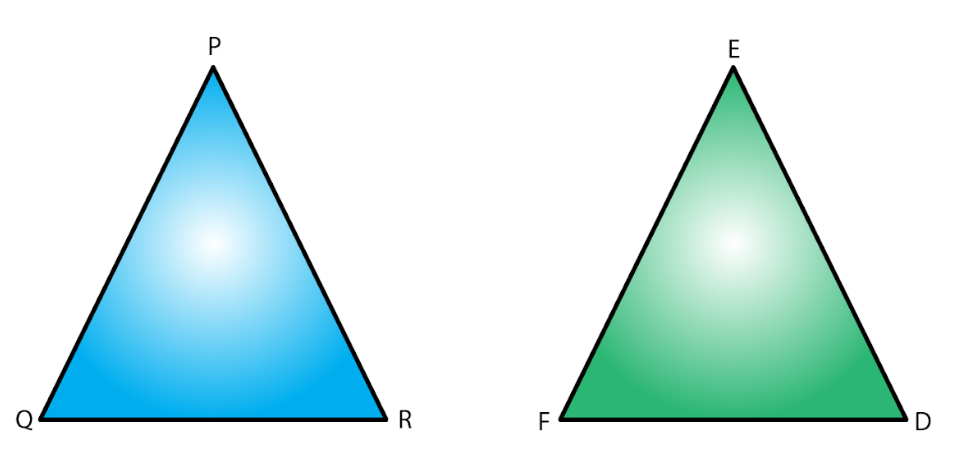(i) PR = ED

Since the corresponding sides of congruent triangles are equal.

(ii) ∠QPR = ∠FED

Since the corresponding angles of congruent triangles are equal.

5. Triangles ABC and PQR are both isosceles with AB = AC and PO = PR respectively. If also, AB = PQ and BC = QR, are the two triangles congruent? Which condition do you use?

It ∠B = 50°, what is the measure of ∠R?

Solution:

Given that AB = AC in isosceles ΔABC

And PQ = PR in isosceles ΔPQR.

Also given that AB = PQ and QR = BC.

Therefore, AC = PR (AB = AC, PQ = PR and AB = PQ)

Hence, ΔABC ≅ ΔPQR

Now

∠ABC = ∠PQR (Since triangles are congruent)

However, ΔPQR is isosceles.

Therefore, ∠PRQ = ∠PQR = ∠ABC = 50o

6. ABC and DBC are both isosceles triangles on a common base BC such that A and D lie on the same side of BC. Are triangles ADB and ADC congruent? Which condition do you use? If ∠BAC = 40° and ∠BDC = 100°, then find ∠ADB.

Solution:

Given ABC and DBC are both isosceles triangles on a common base BC

∠ABC + ∠BCA + ∠BAC = 180o (Angle sum property)

Since ΔABC is an isosceles triangle,

∠ABC = ∠BCA

∠ABC +∠ABC + 40o = 180o

2 ∠ABC = 180o– 40o = 140o

∠ABC = 140o/2 = 70o

∠DBC + ∠ BCD + ∠ BDC = 180o (Angle sum property)

Since ΔABC is an isosceles triangle, ∠ DBC = ∠BCD

∠DBC + ∠DBC + 100= 180o

2 ∠DBC = 180°– 100o = 80o

∠DBC = 80o/2 = 40o

30o + 20o + ∠ADB = 180o (∠ADB = ∠ABC – ∠DBC),

7. Δ ABC and ΔABD are on a common base AB, and AC = BD and BC = AD as shown in Fig. 18. Which of the following statements is true?

(i) ΔABC ≅ ΔABD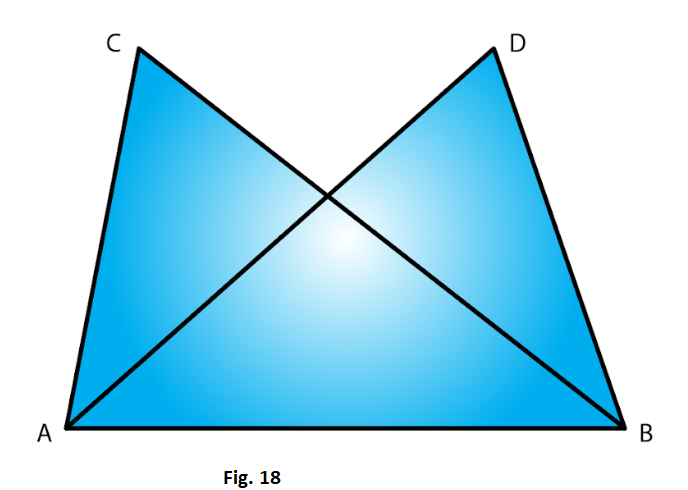Solution:

In ΔABC and ΔBAD we have,

AC = BD (given)

And AB = BA (corresponding parts of congruent triangles)

Therefore by SSS criterion of congruency, ΔABC ≅ ΔBAD

Therefore option (iii) is true.

8. In Fig. 19, ΔABC is isosceles with AB = AC, D is the mid-point of base BC.

(ii) State the three pairs of matching parts you use to arrive at your answer.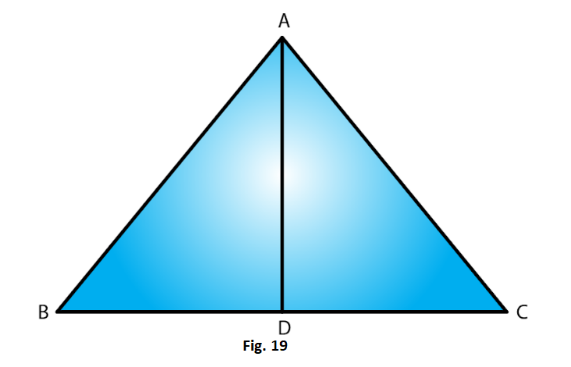Solution:

(i) Given that AB = AC.

Also since D is the midpoint of BC, BD = DC

Therefore by SSS condition,

(ii)We have used AB, AC; BD, DC and AD, DA

9. In fig. 20, ΔABC is isosceles with AB = AC. State if ΔABC ≅ ΔACB. If yes, state three relations that you use to arrive at your answer.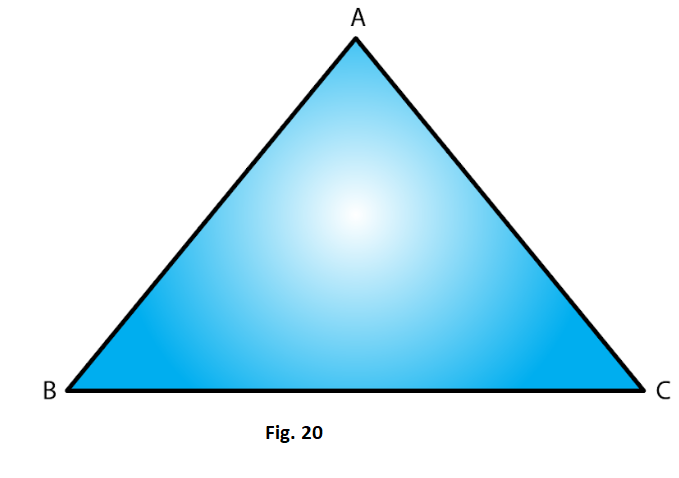Solution:

Given that ΔABC is isosceles with AB = AC

SSS criterion is two triangles are congruent, if the three sides of triangle are respectively equal to the three sides of the other triangle.

ΔABC ≅ ΔACBby SSS condition.

Since, ABC is an isosceles triangle, AB = BC, BC = CB and AC = AB

10. Triangles ABC and DBC have side BC common, AB = BD and AC = CD. Are the two triangles congruent? State in symbolic form, which congruence do you use? Does ∠ABD equal ∠ACD? Why or why not?

Solution: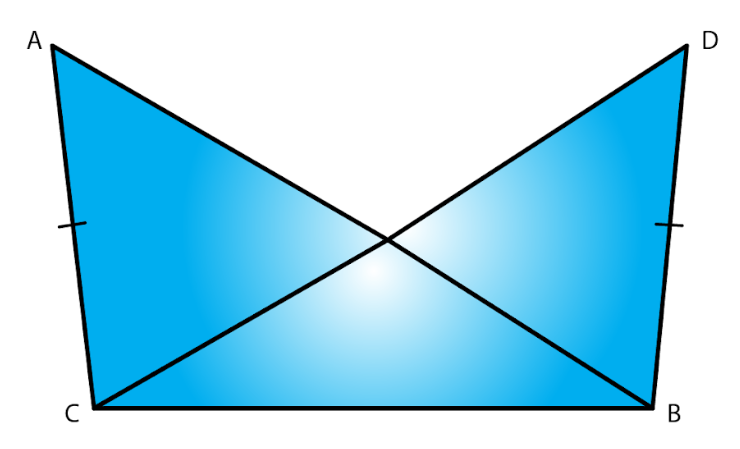Yes, congruent because given that ABC and DBC have side BC common, AB = BD and AC = CD

Also from the above data we can say

By SSS criterion of congruency, ΔABC ≅ ΔDBC

No, ∠ABD and ∠ACD are not equal because AB not equal to AC

Exercise 16.3 Page No: 16.14

1. By applying SAS congruence condition, state which of the following pairs (Fig. 28) of triangle are congruent. State the result in symbolic form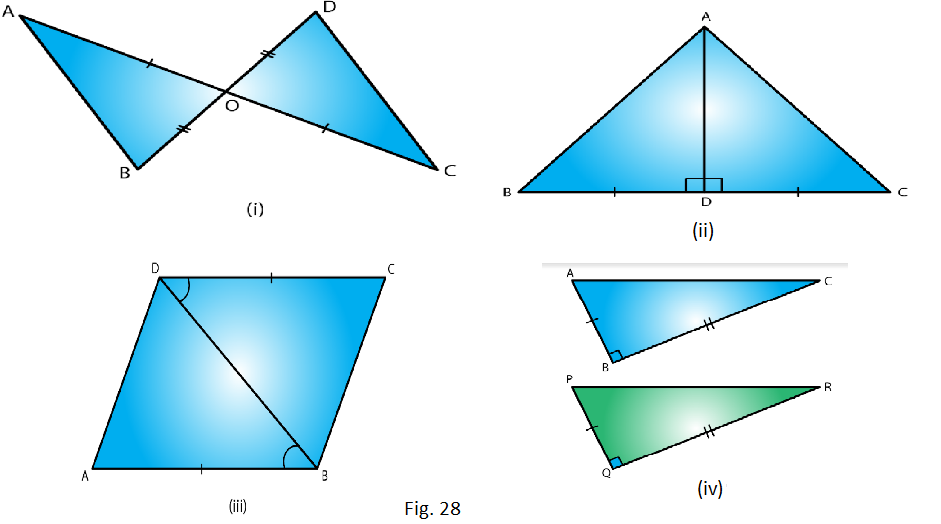Solution:

(i) From the figure we have OA = OC and OB = OD and

∠AOB = ∠COD which are vertically opposite angles.

Therefore by SAS condition, ΔAOC ≅ΔBOD

(ii) From the figure we have BD = DC

(iii) From the figure we have AB = DC

∠ABD = ∠CDB and

Therefore, by SAS condition, ΔABD ≅ΔCBD

(iv) We have BC = QR

ABC = PQR = 90o

And AB = PQ

Therefore, by SAS condition, ΔABC≅ ΔPQR.

2. State the condition by which the following pairs of triangles are congruent.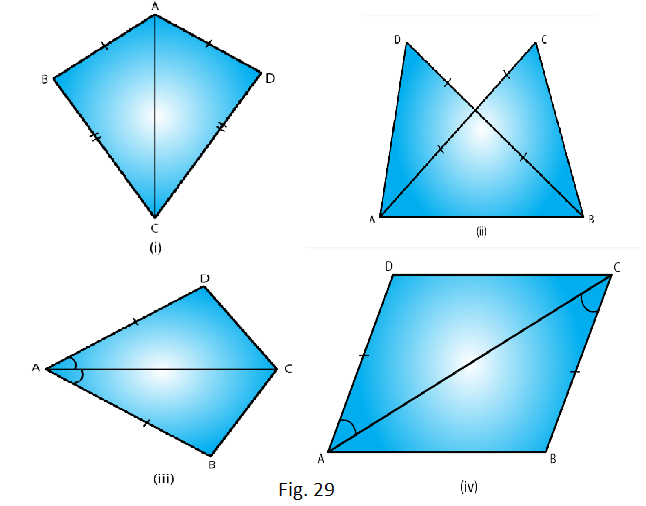Solution:

BC = CD and AC = CA

Therefore by SSS condition, ΔABC≅ ΔADC

(ii) AC = BD

AD = BC and AB = BA

Therefore, by SSS condition, ΔABD ≅ ΔADC

∠BAC = ∠DAC and

Therefore by SAS condition, ΔBAC ≅ ΔBAC

∠DAC = ∠BCA and

Therefore, by SAS condition, ΔABC ≅ ΔADC

3. In fig. 30, line segments AB and CD bisect each other at O. Which of the following statements is true?

(i) ΔAOC ≅ ΔDOB

(ii) ΔAOC ≅ ΔBOD

(iii) ΔAOC ≅ ΔODB

State the three pairs of matching parts, you have used to arrive at the answer.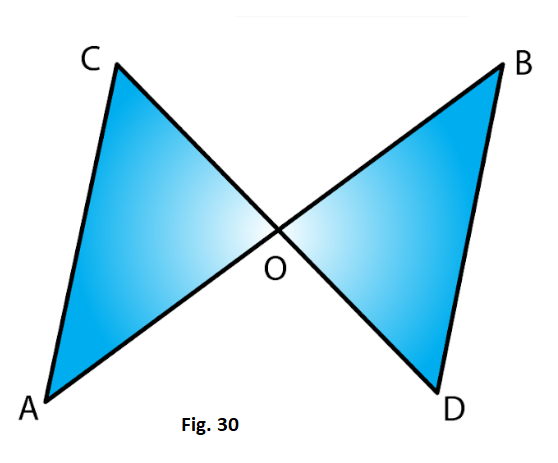Solution:

From the figure we have,

And, CO = OD

Also, AOC = BOD

Therefore, by SAS condition, ΔAOC ≅ ΔBOD

4. Line-segments AB and CD bisect each other at O. AC and BD are joined forming triangles AOC and BOD. State the three equality relations between the parts of the two triangles that are given or otherwise known. Are the two triangles congruent? State in symbolic form, which congruence condition do you use?

Solution:

We have AO = OB and CO = OD

Since AB and CD bisect each other at 0.

Also ∠AOC = ∠BOD

Since they are opposite angles on the same vertex.

Therefore by SAS congruence condition, ΔAOC ≅ ΔBOD

5. ΔABC is isosceles with AB = AC. Line segment AD bisects ∠A and meets the base BC in D.

(ii) State the three pairs of matching parts used to answer (i).

(iii) Is it true to say that BD = DC?

Solution:

(i) We have AB = AC (Given)

Therefore by SAS condition of congruence, ΔABD ≅ ΔACD

(iii) Now, ΔABD≅ΔACD

Therefore by corresponding parts of congruent triangles

BD = DC.

6. In Fig. 31, AB = AD and ∠BAC = ∠DAC.

(i) State in symbolic form the congruence of two triangles ABC and ADC that is true.

(ii) Complete each of the following, so as to make it true:

(a) ∠ABC =

(b) ∠ACD =

(c) Line segment AC bisects ….. And ……..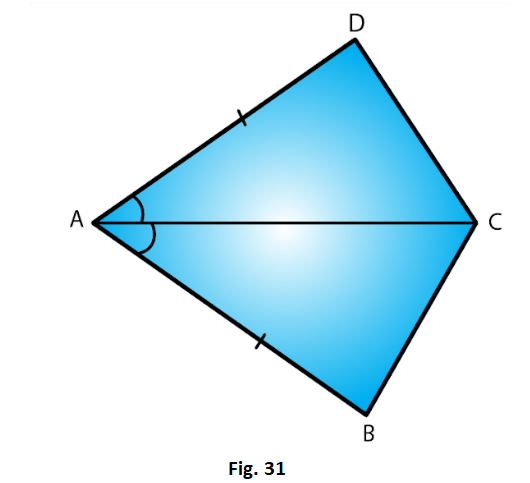Solution:

∠BAC = ∠DAC (given)

AC = CA (common)

Therefore by SAS condition of congruency, ΔABC ≅ ΔADC

ii) ∠ABC = ∠ADC (corresponding parts of congruent triangles)

∠ACD = ∠ACB (corresponding parts of congruent triangles)

7. In fig. 32, AB || DC and AB = DC.

(i) Is ΔACD ≅ ΔCAB?

(ii) State the three pairs of matching parts used to answer (i).

(iii) Which angle is equal to ∠CAD?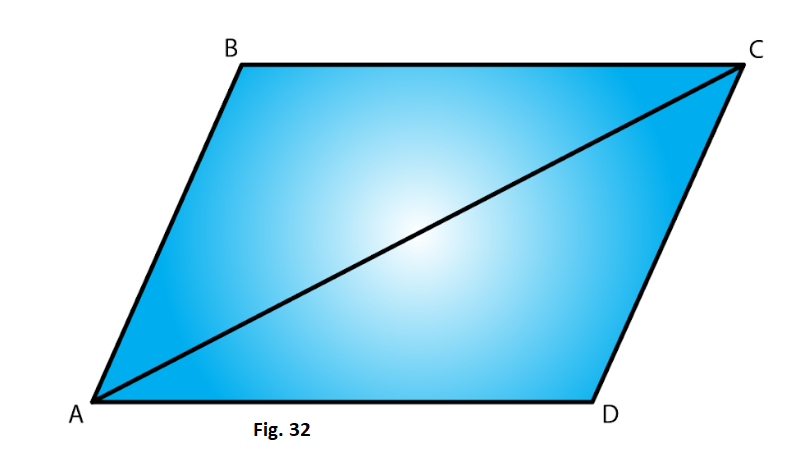Solution:

(i) Yes by SAS condition of congruency, ΔDCA ≅ ΔBAC

(ii) We have used AB = DC, AC = CA and ∠DCA = ∠BAC.

(iii) ∠CAD = ∠ACB since the two triangles are congruent.

(iv) Yes this follows from AD parallel to BC as alternate angles are equal. lf alternate angles are equal the lines are parallel

Exercise 16.4 Page No: 16.19

1. Which of the following pairs of triangle are congruent by ASA condition?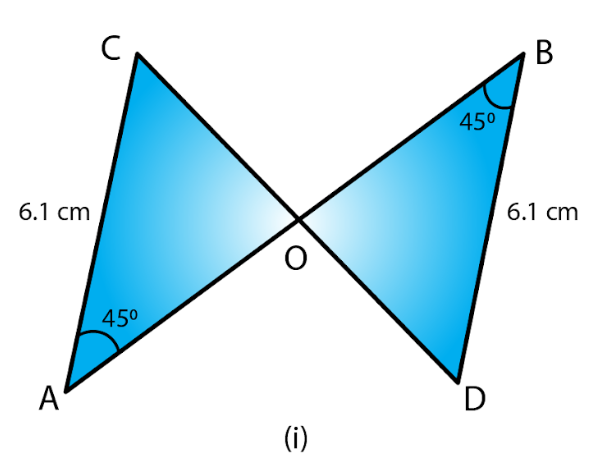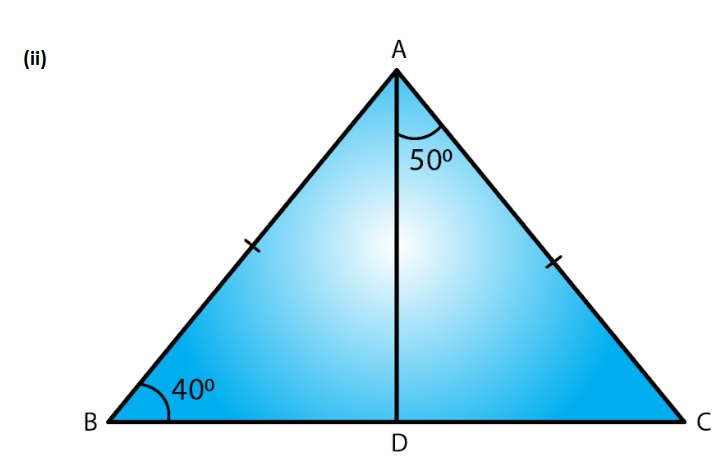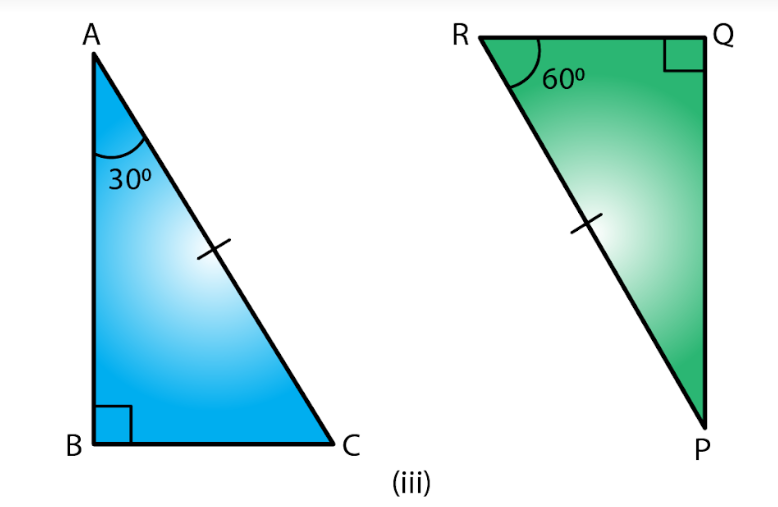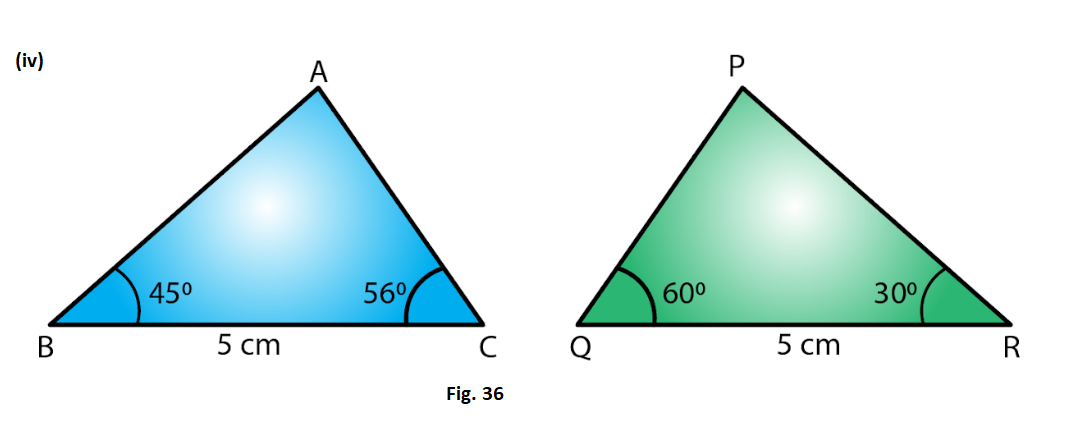Solution:

(i) We have,

Since ∠ABO = ∠CDO = 45o and both are alternate angles, AB parallel to DC, ∠BAO = ∠DCO (alternate angle, AB parallel to CD and AC is a transversal line)

∠ABO = ∠CDO = 45o (given in the figure) Also,

AB = DC (Given in the figure)

Therefore, by ASA ΔAOB ≅ ΔDOC

(ii) In ABC,

Now AB =AC (Given)

∠ABD = ∠ACD = 40o (Angles opposite to equal sides)

∠ABD + ∠ACD + ∠BAC = 180o (Angle sum property)

40o + 40o + ∠BAC = 180o

∠BAC =180o – 80o =100o

∠BAD = ∠BAC – ∠DAC = 100o – 50o = 50o

Therefore, by ASA, ΔABD ≅ ΔADC

(iii) In Δ ABC,

∠A + ∠B + ∠C = 180o (Angle sum property)

∠C = 180o– ∠A – ∠B

∠C = 180o – 30o – 90o = 60o

In PQR,

∠P + ∠Q + ∠R = 180o (Angle sum property)

∠P = 180o – ∠Q – ∠R

∠P = 180o – 60o – 90o = 30o

∠BAC = ∠QPR = 30o

∠BCA = ∠PRQ = 60o and AC = PR (Given)

Therefore, by ASA, ΔABC ≅ ΔPQR

(iv) We have only

BC = QR but none of the angles of ΔABC and ΔPQR are equal.

Therefore, ΔABC and Cong ΔPRQ

(ii) State the three pairs of matching parts you have used in (i)

(iii) Is it true to say that BD = DC?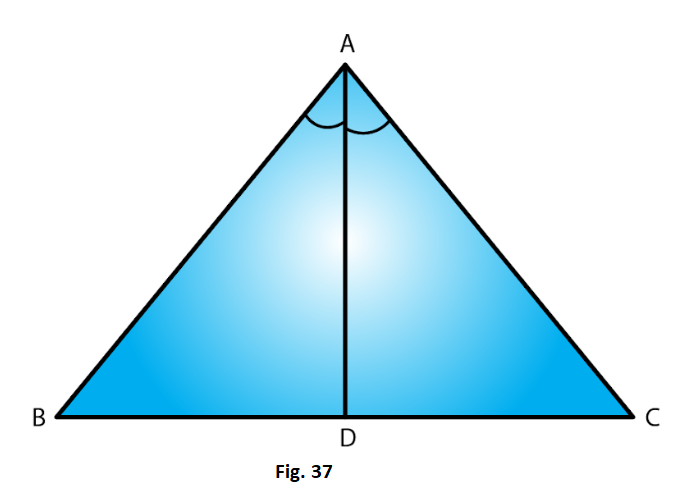Solution:

3. Draw any triangle ABC. Use ASA condition to construct other triangle congruent to it.

Solution: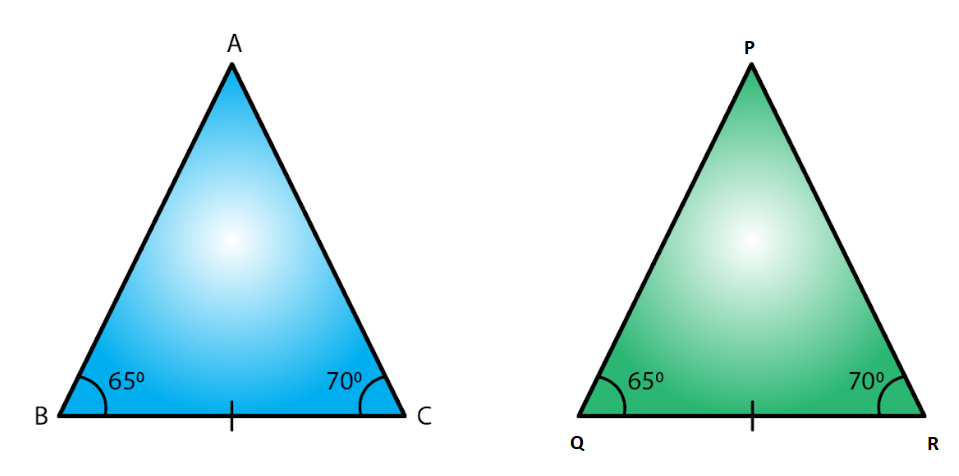We have drawn

Δ ABC with ∠ABC = 65o and ∠ACB = 70o

We now construct ΔPQR ≅ ΔABC has ∠PQR = 65o and ∠PRQ = 70o

Also we construct ΔPQR such that BC = QR

Therefore by ASA the two triangles are congruent

4. In Δ ABC, it is known that ∠B = C. Imagine you have another copy of Δ ABC

(i) Is ΔABC ≅ ΔACB

(ii) State the three pairs of matching parts you have used to answer (i).

(iii) Is it true to say that AB = AC?

Solution: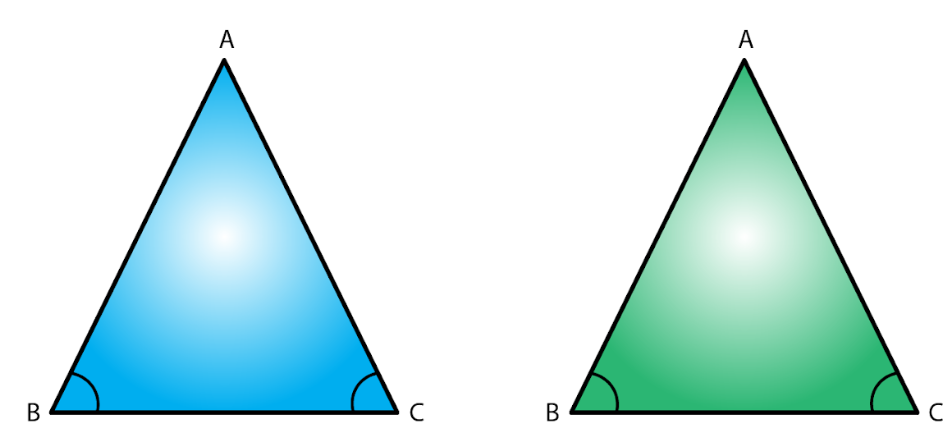(i) Yes ΔABC ≅ ΔACB

(ii) We have used ∠ABC = ∠ACB and ∠ACB = ∠ABC again.

Also BC = CB

(iii) Yes it is true to say that AB = AC since ∠ABC = ∠ACB.

5. In Fig. 38, AX bisects ∠BAC as well as ∠BDC. State the three facts needed to ensure that ΔACD ≅ ΔABD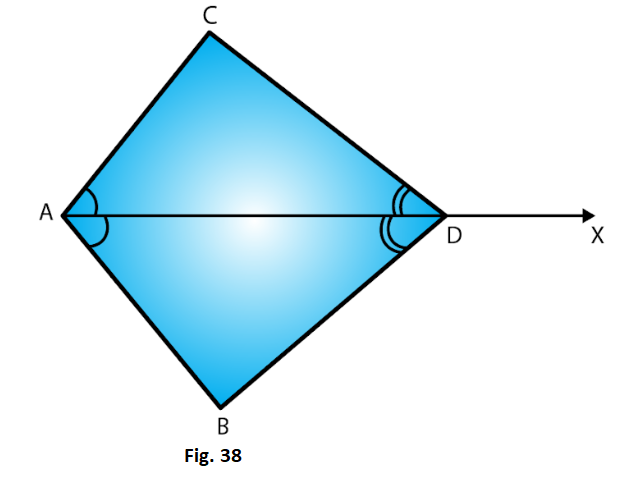Solution:

As per the given conditions,

Therefore, by ASA, ΔACD ≅ ΔABD

6. In Fig. 39, AO = OB and ∠A = ∠B.

(i) Is ΔAOC ≅ ΔBOD

(ii) State the matching pair you have used, which is not given in the question.

(iii) Is it true to say that ∠ACO = ∠BDO?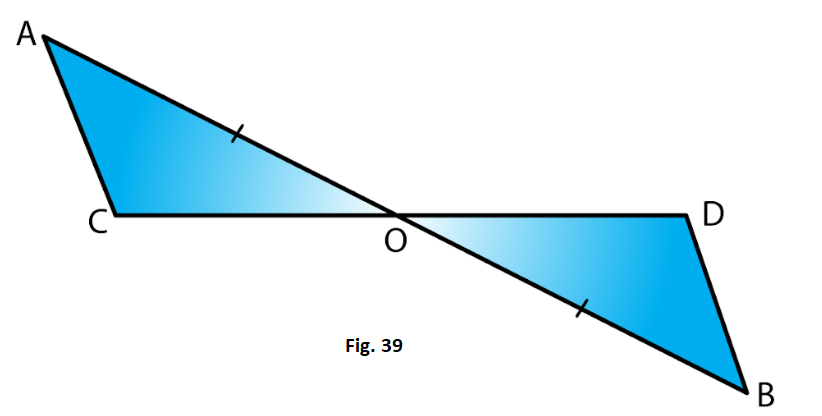Solution:

We have

∠OAC = ∠OBD,

AO = OB

Also, ∠AOC = ∠BOD (Opposite angles on same vertex)

Therefore, by ASA ΔAOC ≅ ΔBOD

Exercise 16.5 Page No: 16.23

1. In each of the following pairs of right triangles, the measures of some part are indicated alongside. State by the application of RHS congruence conditions which are congruent, and also state each result in symbolic form. (Fig. 46)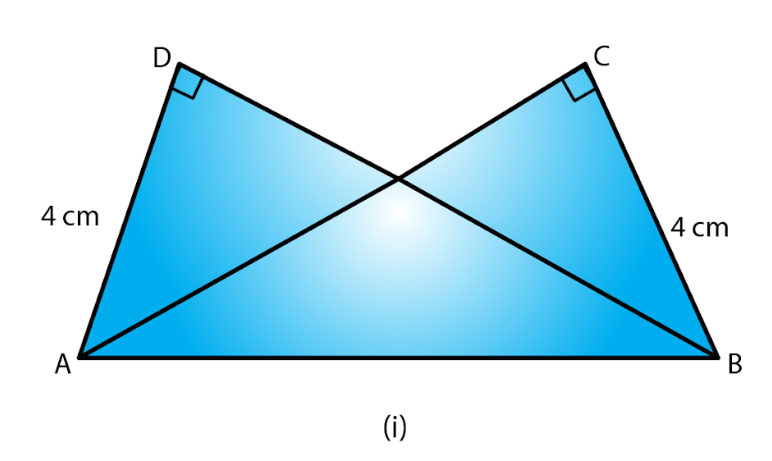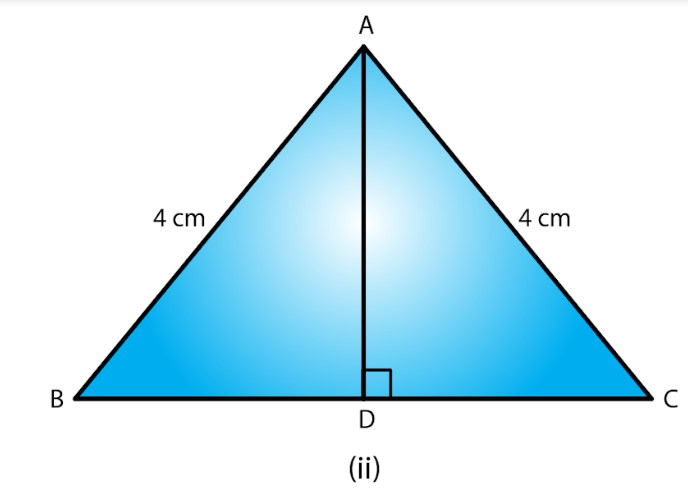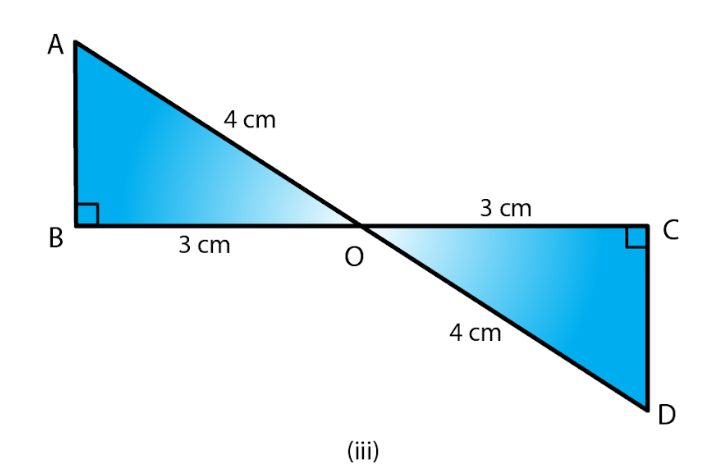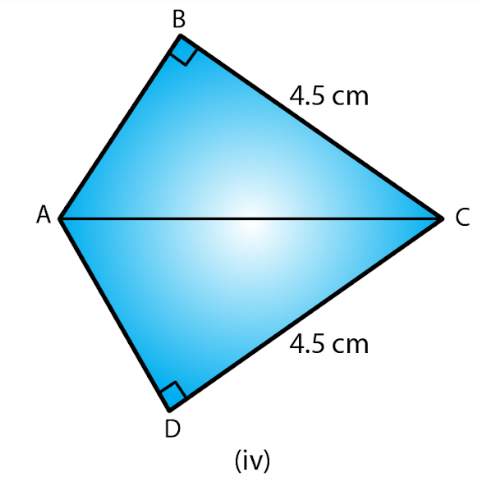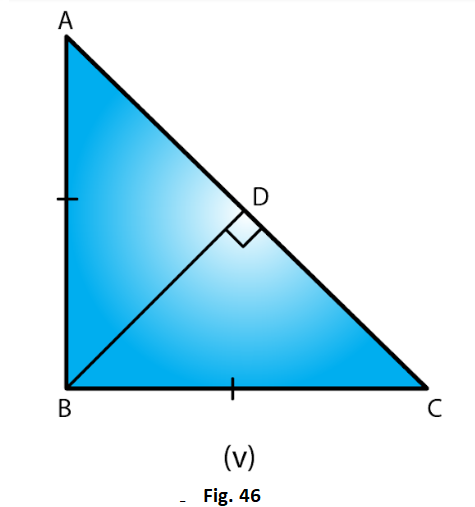Solution:

(i) ∠ADC = ∠BCA = 90o

AD = BC and hypotenuse AB = hypotenuse AB

Therefore, by RHS ΔADB ≅ ΔACB

Hypotenuse AC = hypotenuse AB (Given)

∠ADB = 180o – 90o = 90o

(iii) Hypotenuse AO = hypotenuse DO

BO = CO

∠B = ∠C = 90o

Therefore, by RHS, ΔAOB≅ΔDOC

(iv) Hypotenuse AC = Hypotenuse CA

BC = DC

Therefore, by RHS, ΔABC ≅ ΔADC

(v) BD = DB

Hypotenuse AB = Hypotenuse BC, as per the given figure,

∠BDA + ∠BDC = 180o

∠BDA + 90o = 180o

∠BDA= 180o – 90o = 90o

∠BDA = ∠BDC = 90o

Therefore, by RHS, ΔABD ≅ ΔCBD

2. Δ ABC is isosceles with AB = AC. AD is the altitude from A on BC.

(i) Is ΔABD ≅ ΔACD?

(ii) State the pairs of matching parts you have used to answer (i).

(iii) Is it true to say that BD = DC?

Solution:

(i) Yes, ΔABD ≅ ΔACD by RHS congruence condition.

(ii) We have used Hypotenuse AB = Hypotenuse AC

(iii)Yes, it is true to say that BD = DC (corresponding parts of congruent triangles)

Since we have already proved that the two triangles are congruent.

3. ΔABC is isosceles with AB = AC. Also. AD ⊥ BC meeting BC in D. Are the two triangles ABD and ACD congruent? State in symbolic form. Which congruence condition do you use? Which side of ADC equals BD? Which angle of Δ ADC equals ∠B?

Solution:

We have AB = AC …… (i)

AD = DA (common) …… (ii)

Therefore, from (i), (ii) and (iii), by RHS congruence condition, ΔABD ≅ ΔACD, the triangles are congruent.

Therefore, BD = CD.

And ∠ABD = ∠ACD (corresponding parts of congruent triangles)

4. Draw a right triangle ABC. Use RHS condition to construct another triangle congruent to it.

Solution: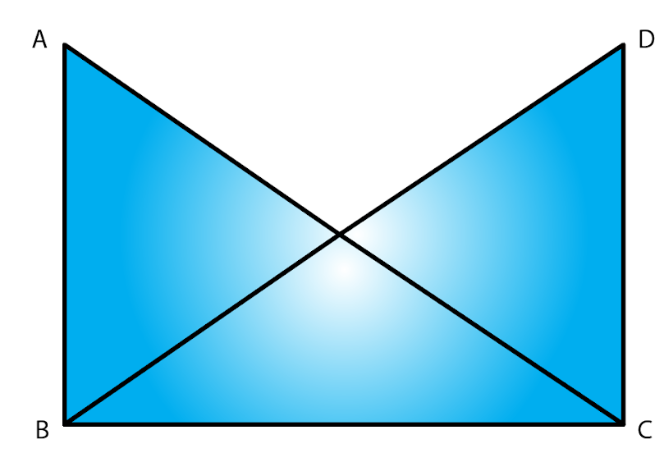Consider

Δ ABC with ∠B as right angle.

We now construct another triangle on base BC, such that ∠C is a right angle and AB = DC

Also, BC = CB

Therefore, BC = CB

Therefore by RHS, ΔABC ≅ ΔDCB

5.In fig. 47, BD and CE are altitudes of Δ ABC and BD = CE.

(i) Is ΔBCD ≅ ΔCBE?

(ii) State the three pairs or matching parts you have used to answer (i)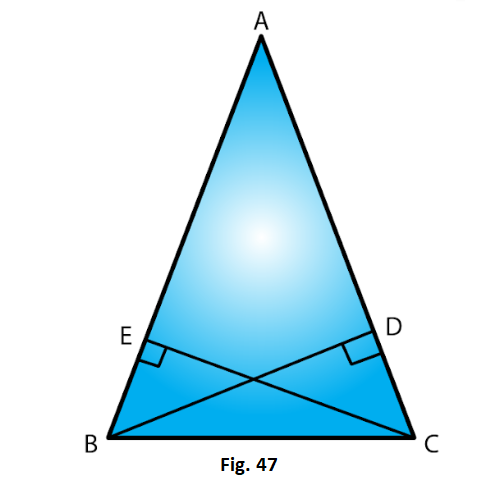Solution:

(i) Yes, ΔBCD ≅ ΔCBE by RHS congruence condition.

(ii) We have used hypotenuse BC = hypotenuse CB

BD = CE (Given in question)

And ∠BDC = ∠CBE = 90o